# RD Sharma Solutions for Class 11 Maths Chapter 1 Sets

## RD Sharma Solutions Class 11 Maths Chapter 1 – Free PDF Download

RD Sharma Solutions for Class 11 Maths Chapter 1- Sets are available here. In this chapter, we start with two undefined (or primitive) terms – “element” and “set”. We assume that the word “set” is synonymous with the words “collection”, “aggregate”, “class” and is composed of elements. The words “element”, “object”, “member” are synonymous. All these topics are discussed by our expert faculty team in RD Sharma Solutions for Class 11 book. Tutors at BYJU’S have designed the solutions in a unique and simple method where any student can understand the topics clearly. PDF consisting of the chapter solutions can be easily downloaded from the links provided below.

Chapter 1- Sets contains eight exercises and the RD Sharma Class 11 Solutions present in this page provide solutions to the questions present in each exercise. Now, let us have a look at the concepts discussed in this chapter.

• Sets and Description of Set.
• Roaster form or tabular form.
• Set-builder form.
• Types of sets.
• Subsets, universal set, power set.
• Venn diagrams.
• Operations on sets.
• Laws of the algebra of sets.
• Some important results on the number of elements in sets.

## Download the Pdf of RD Sharma Solutions for Class 11 Maths Chapter 1 – Sets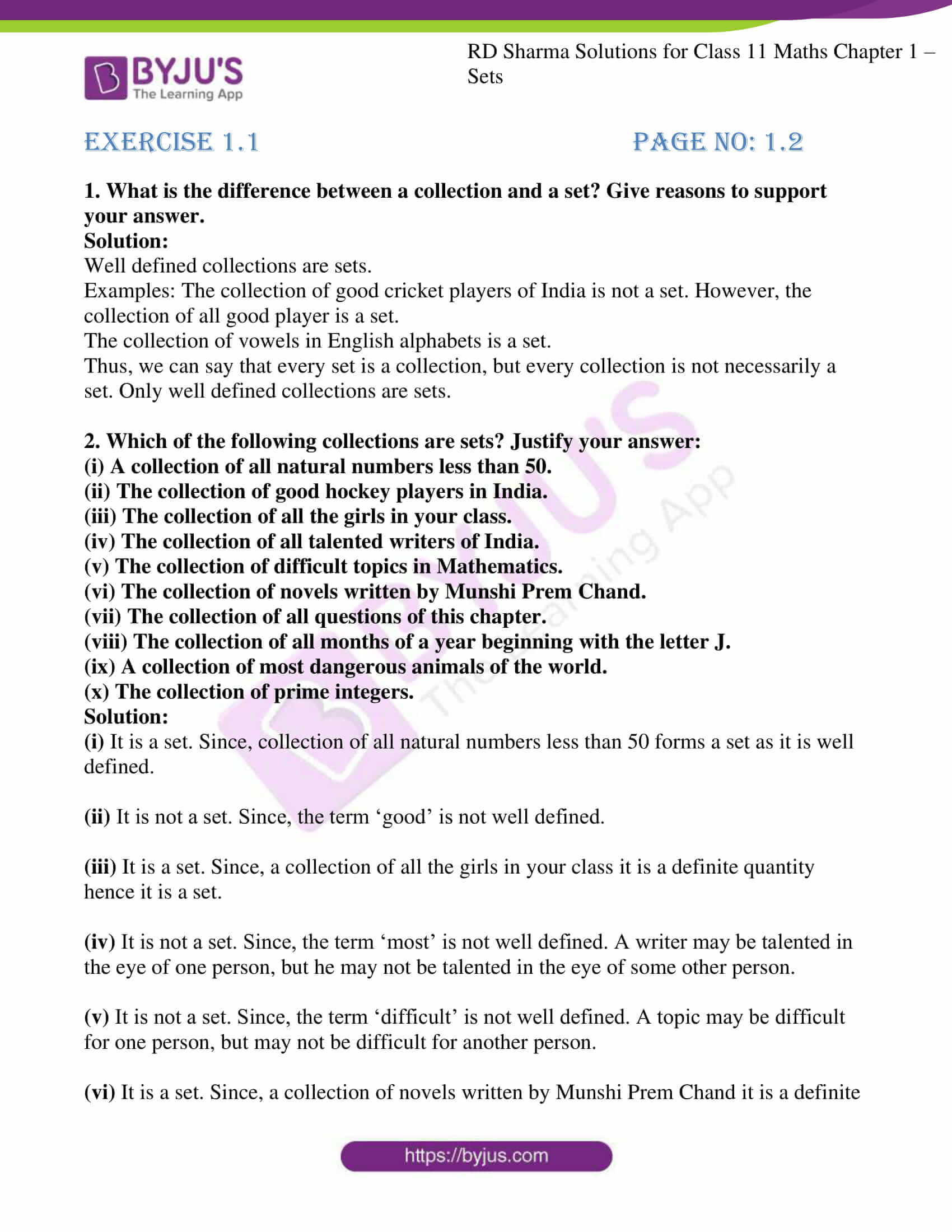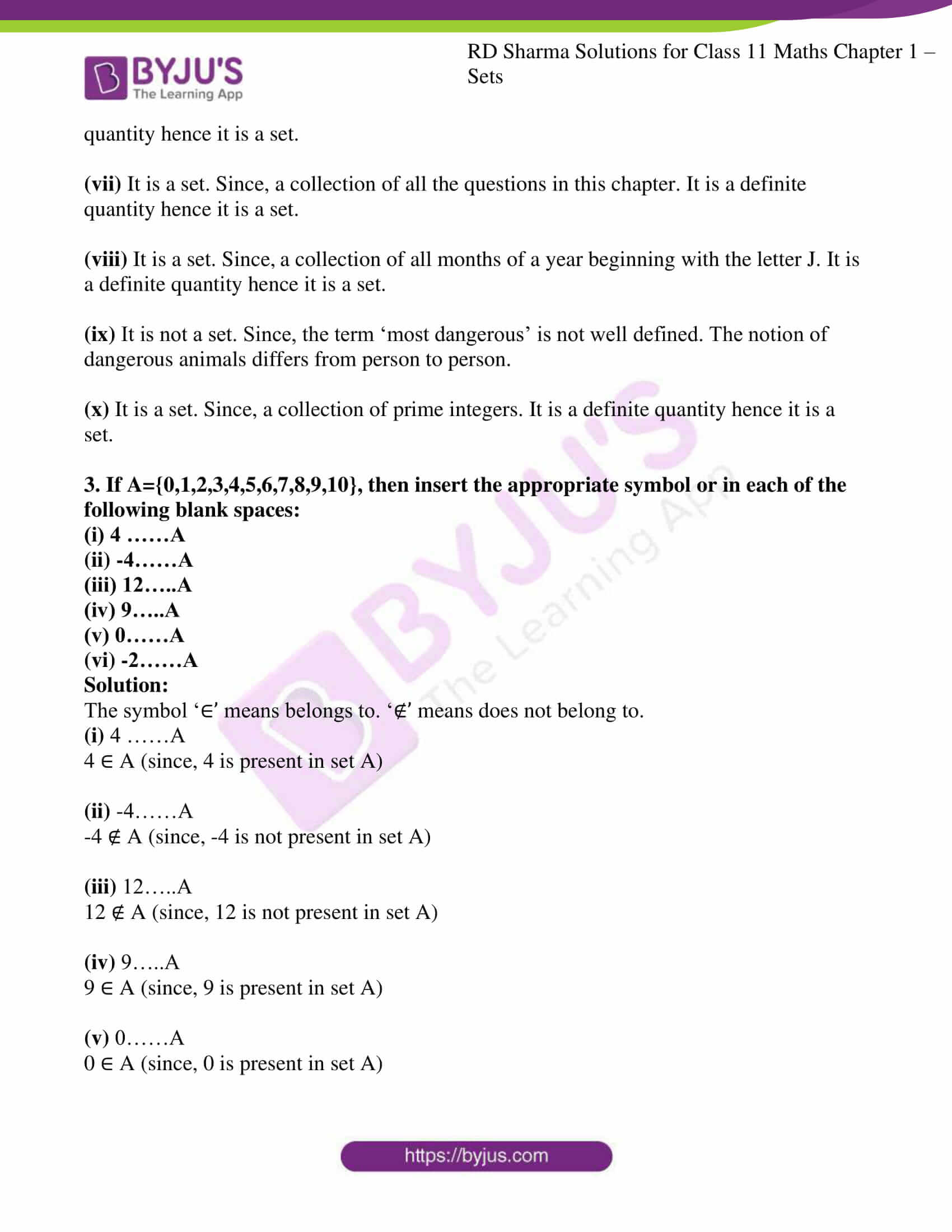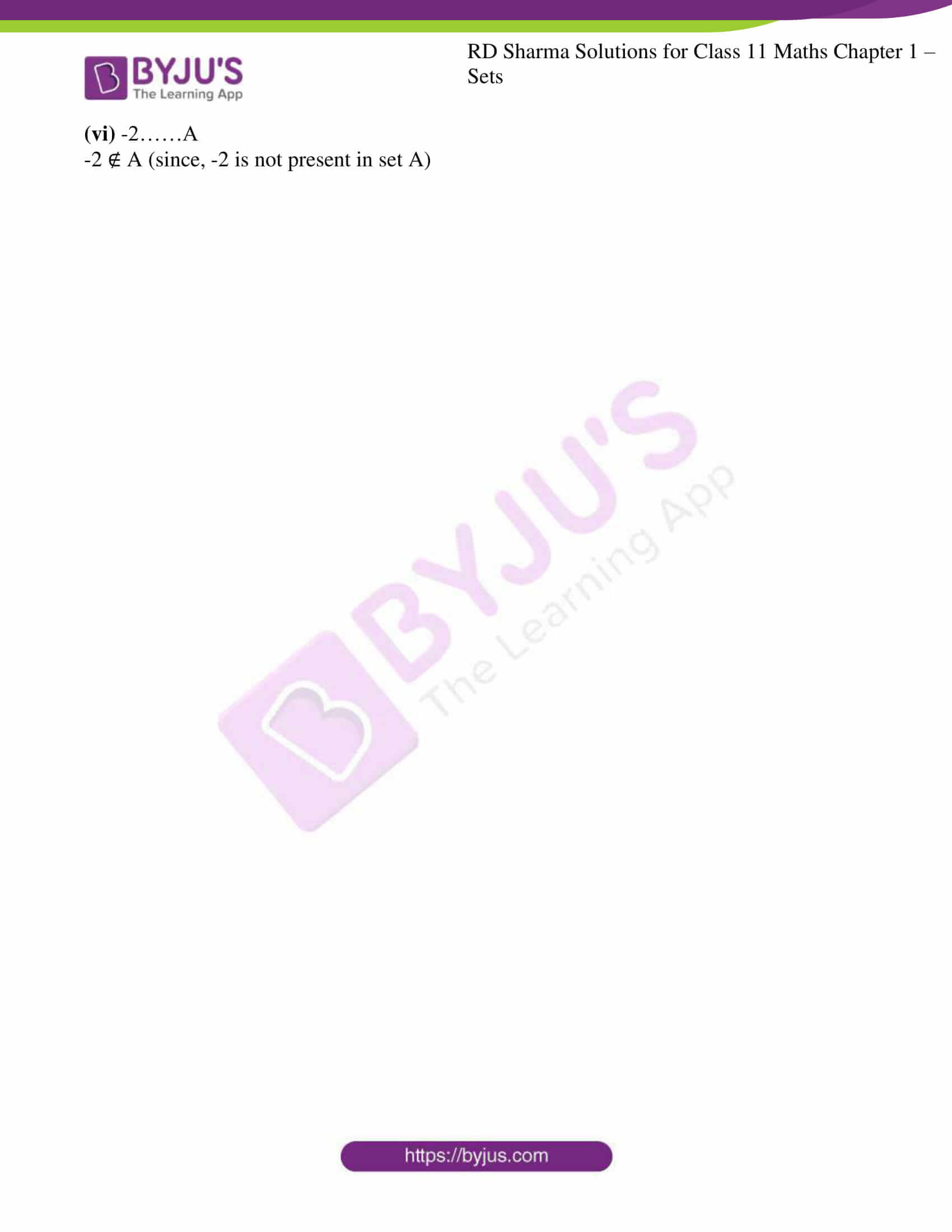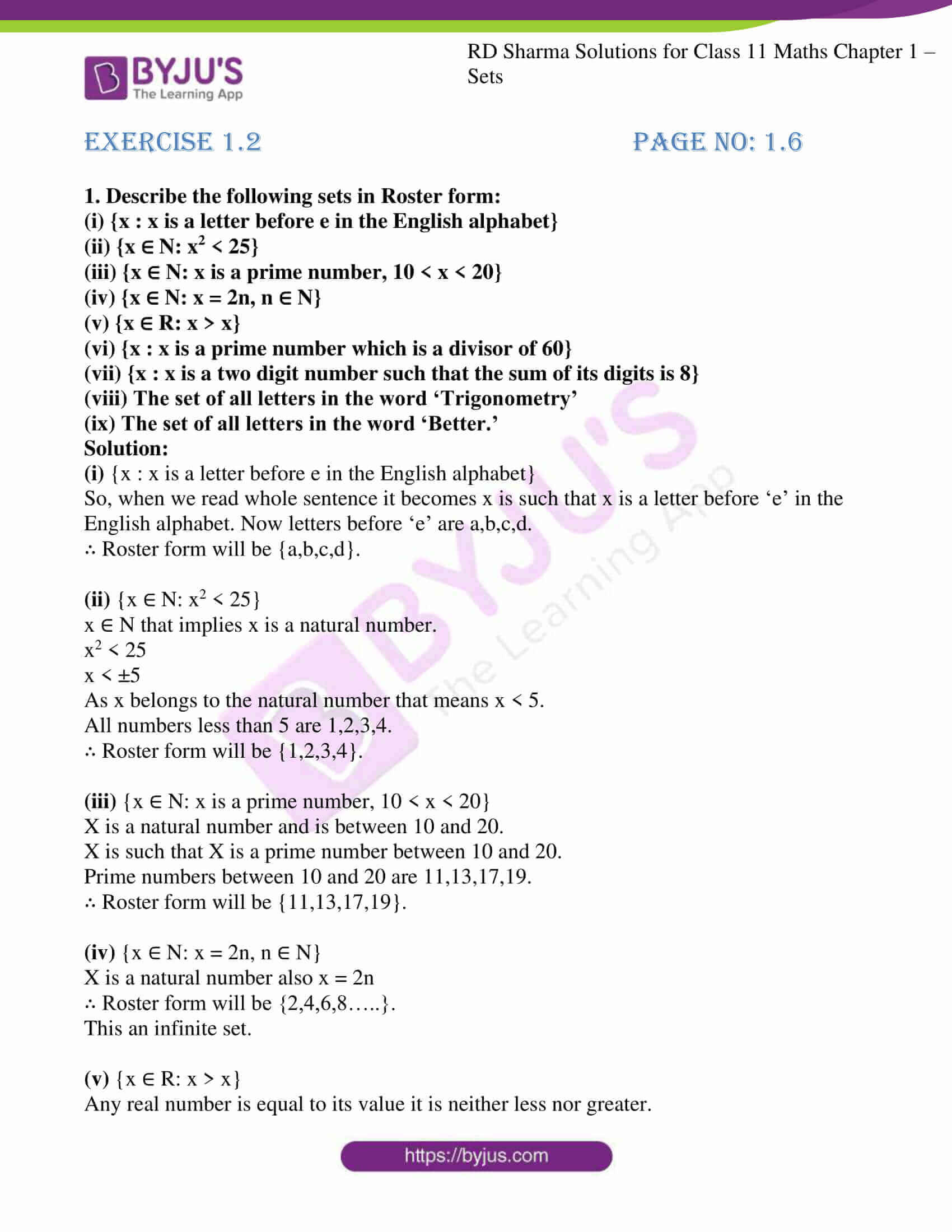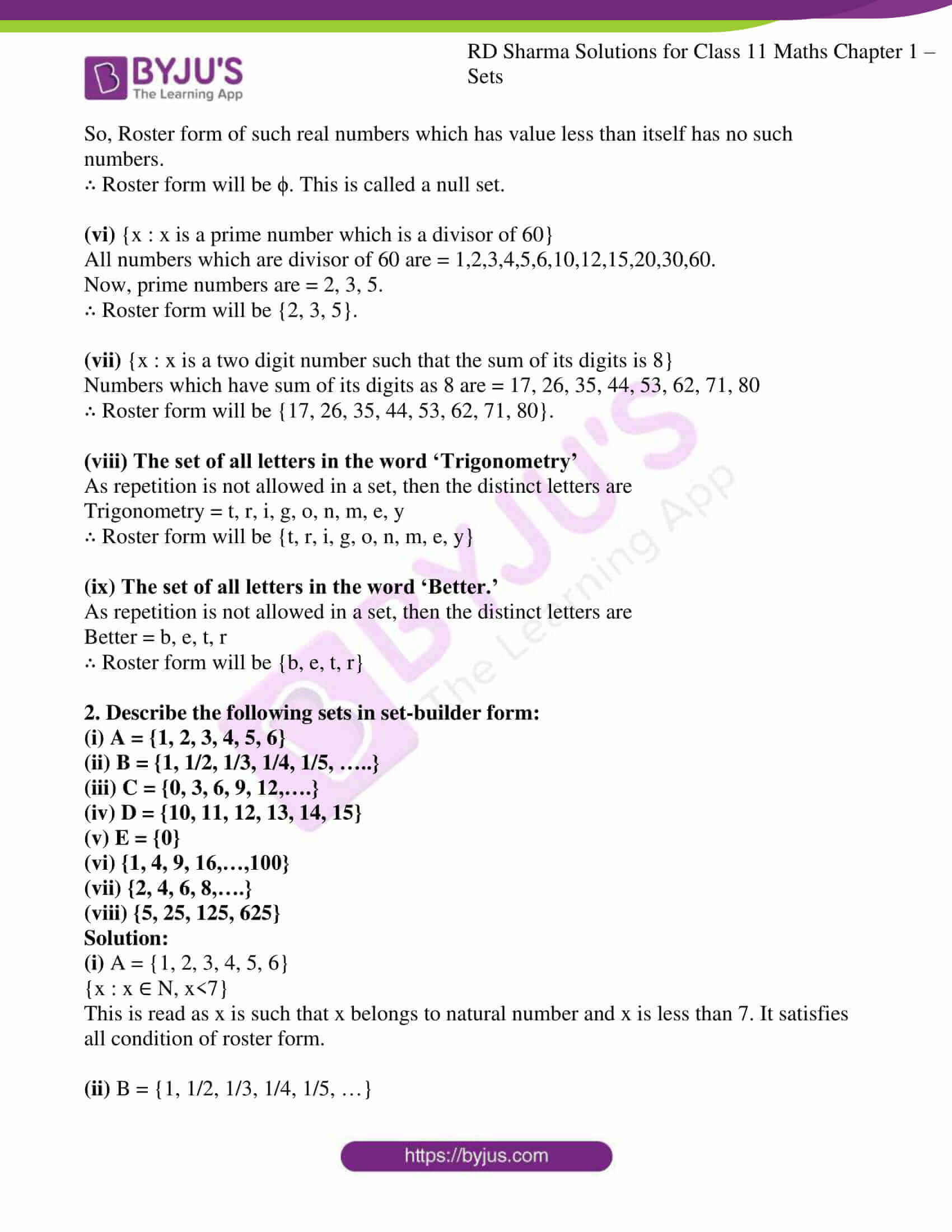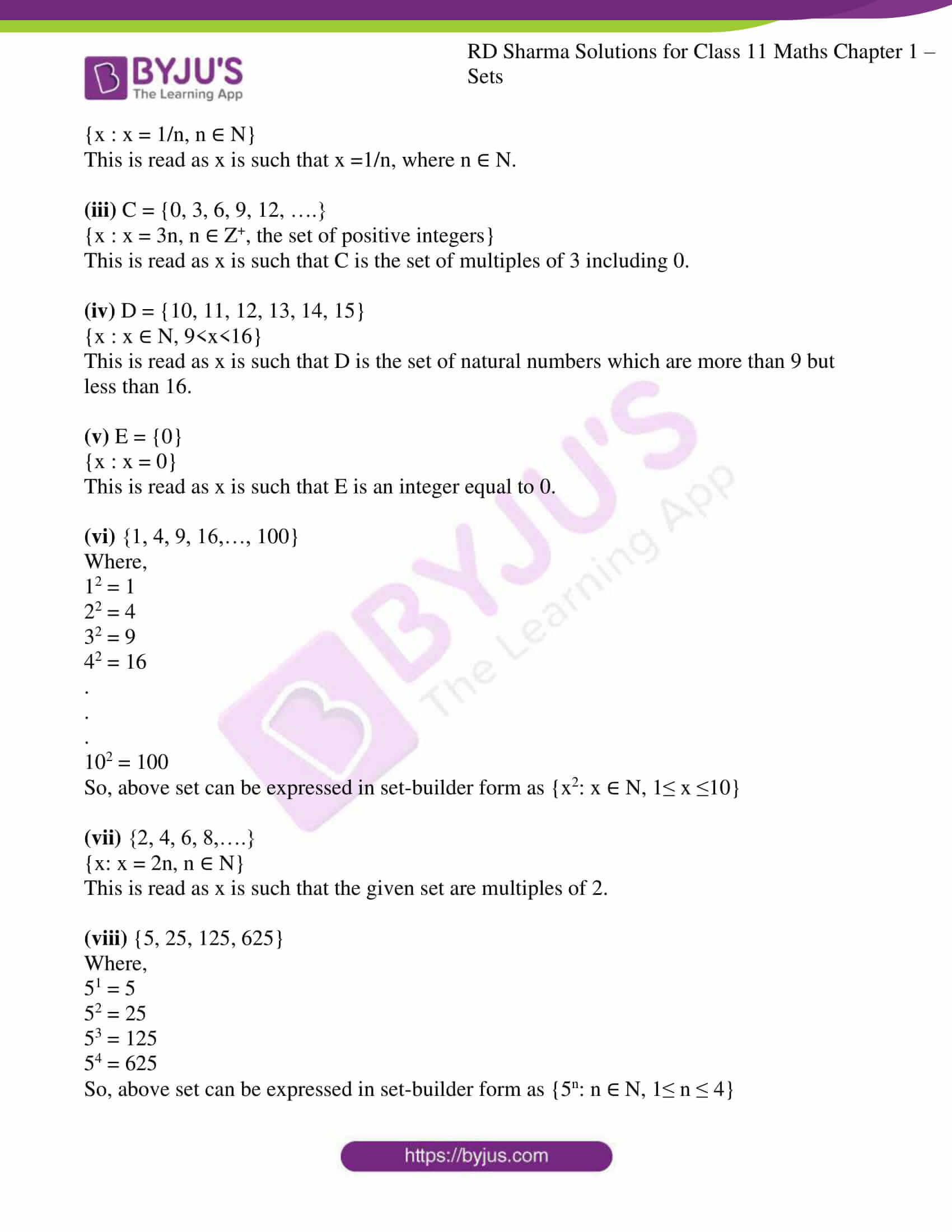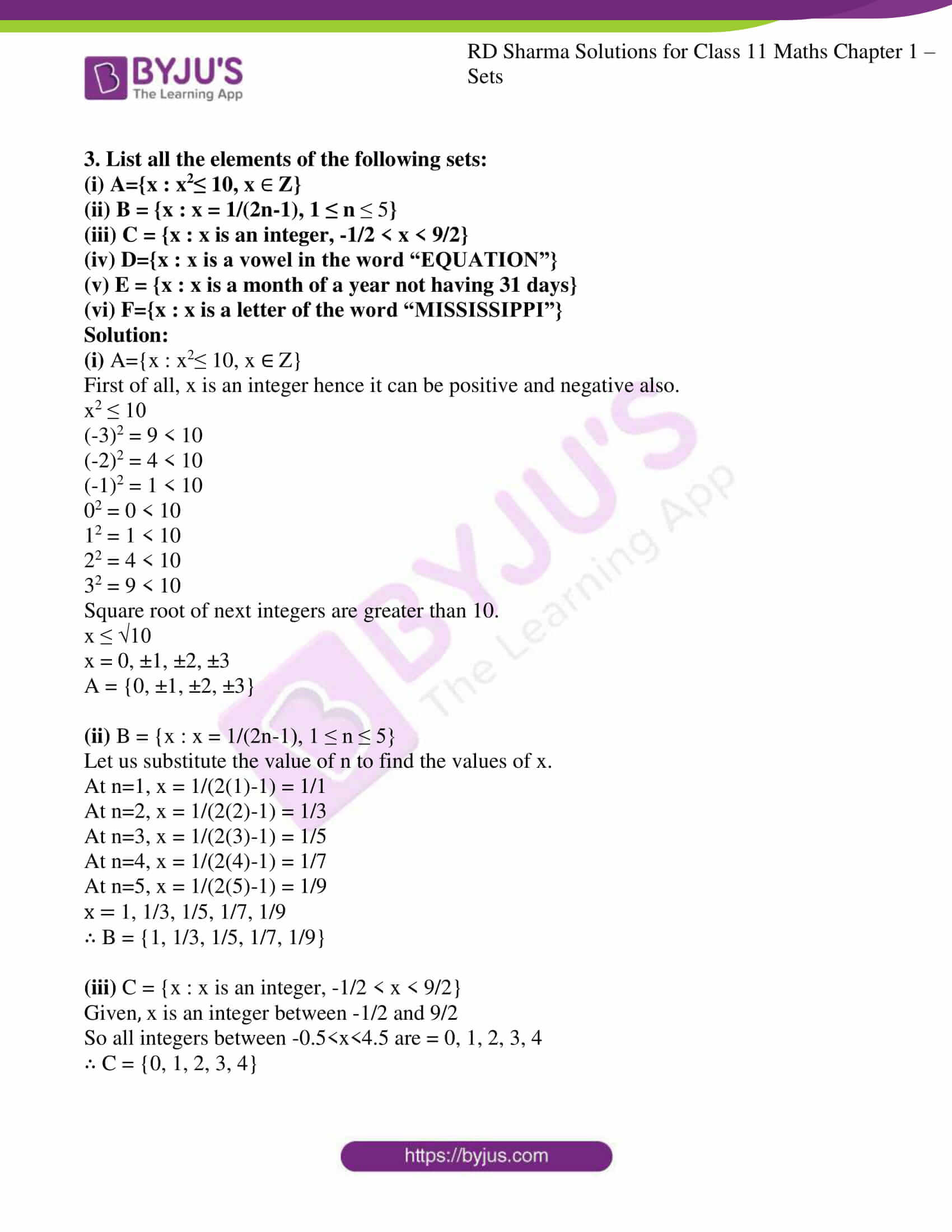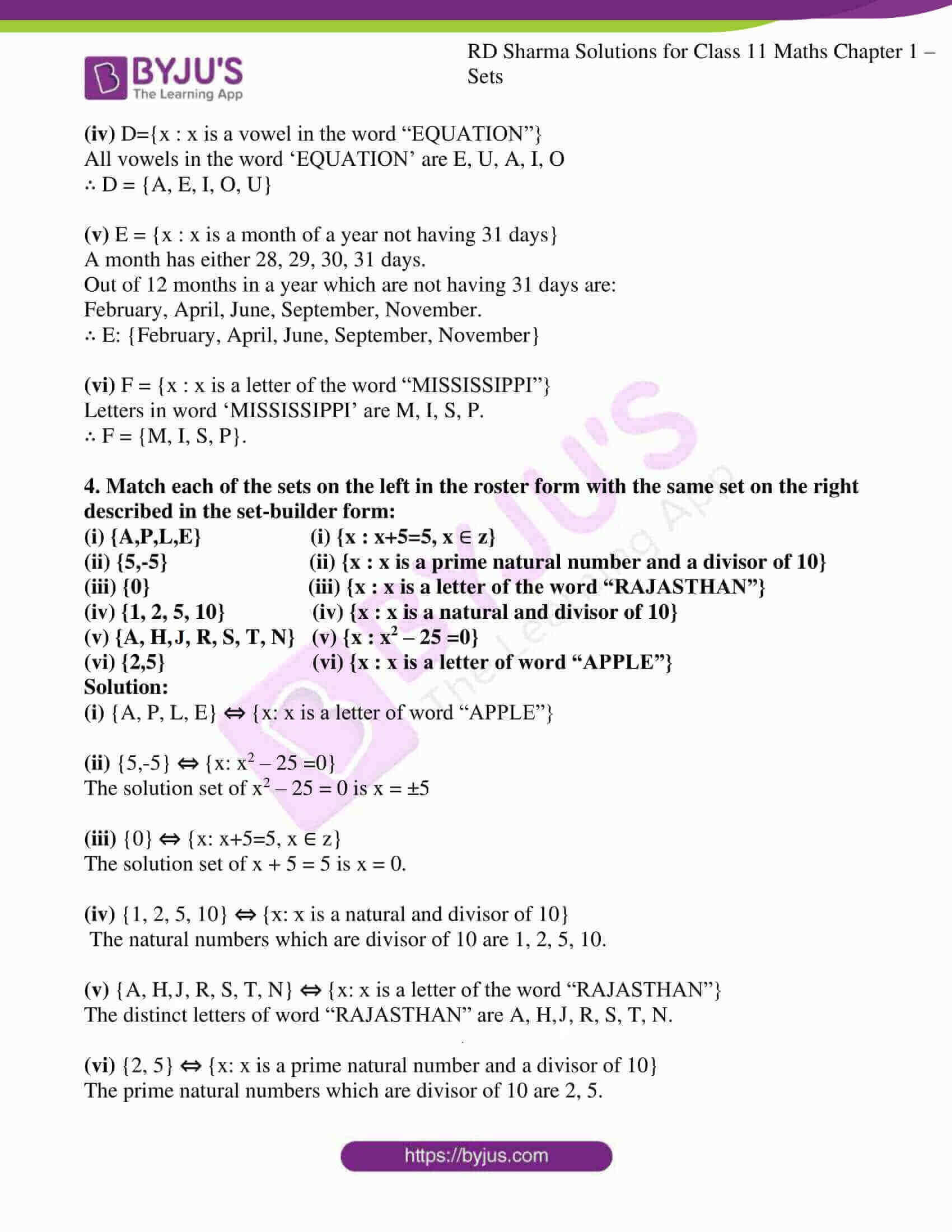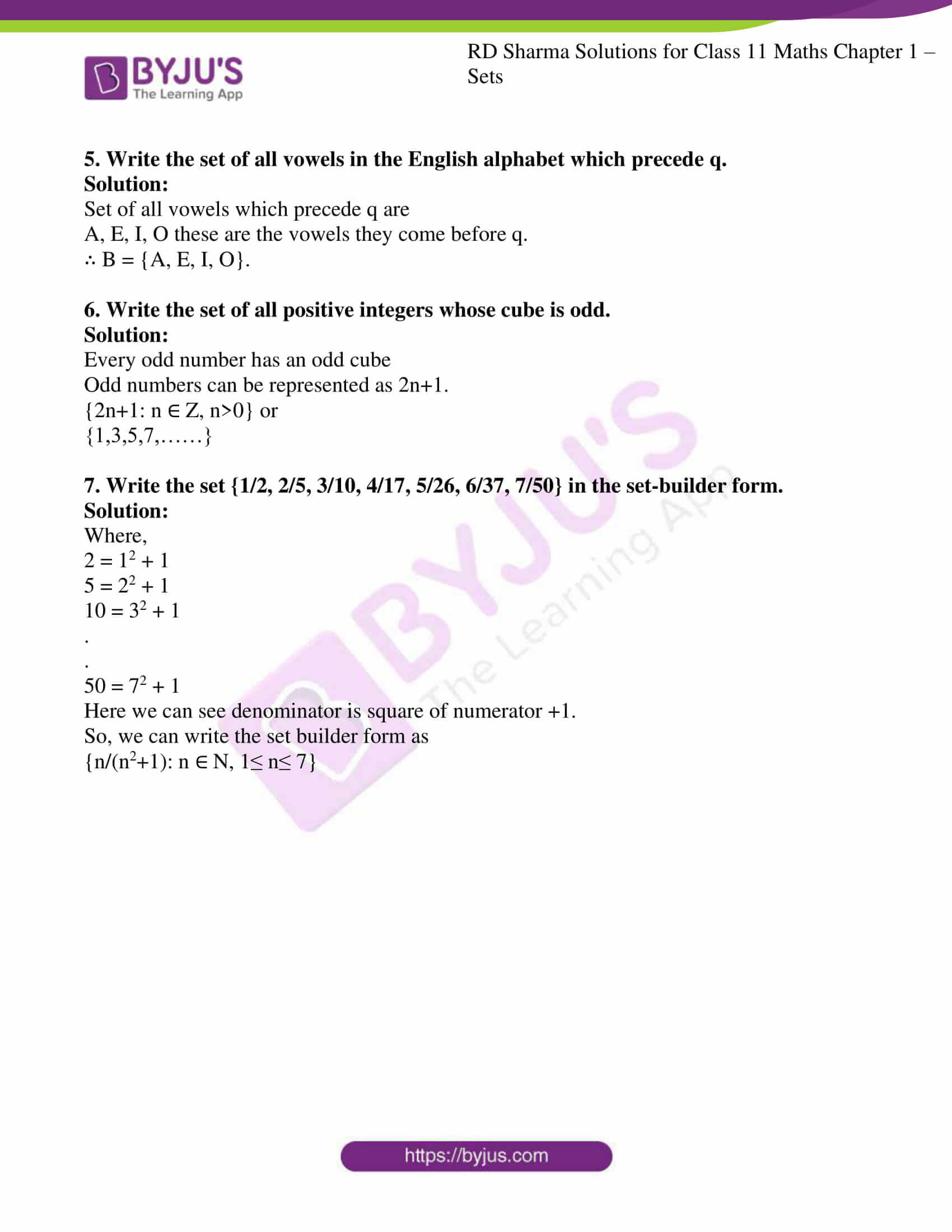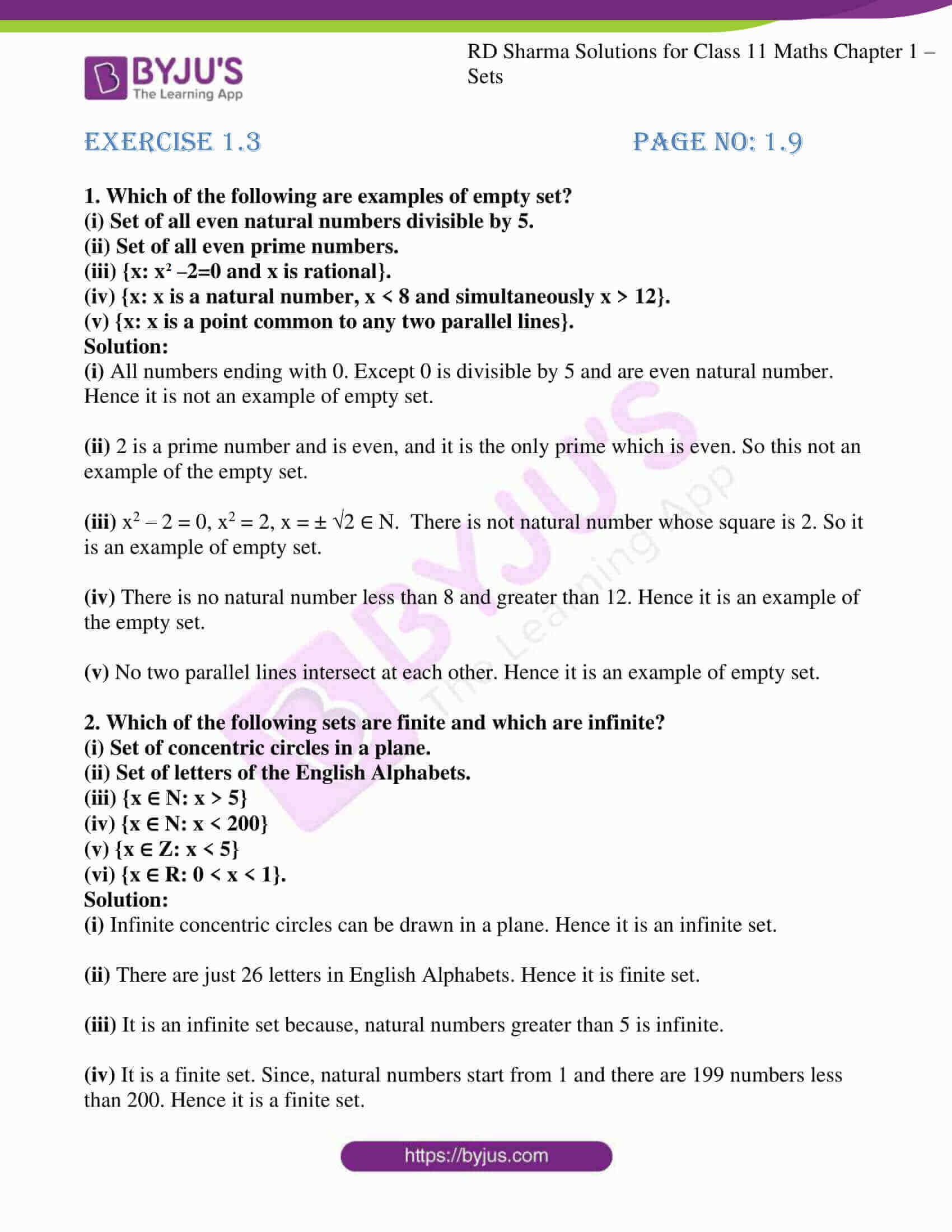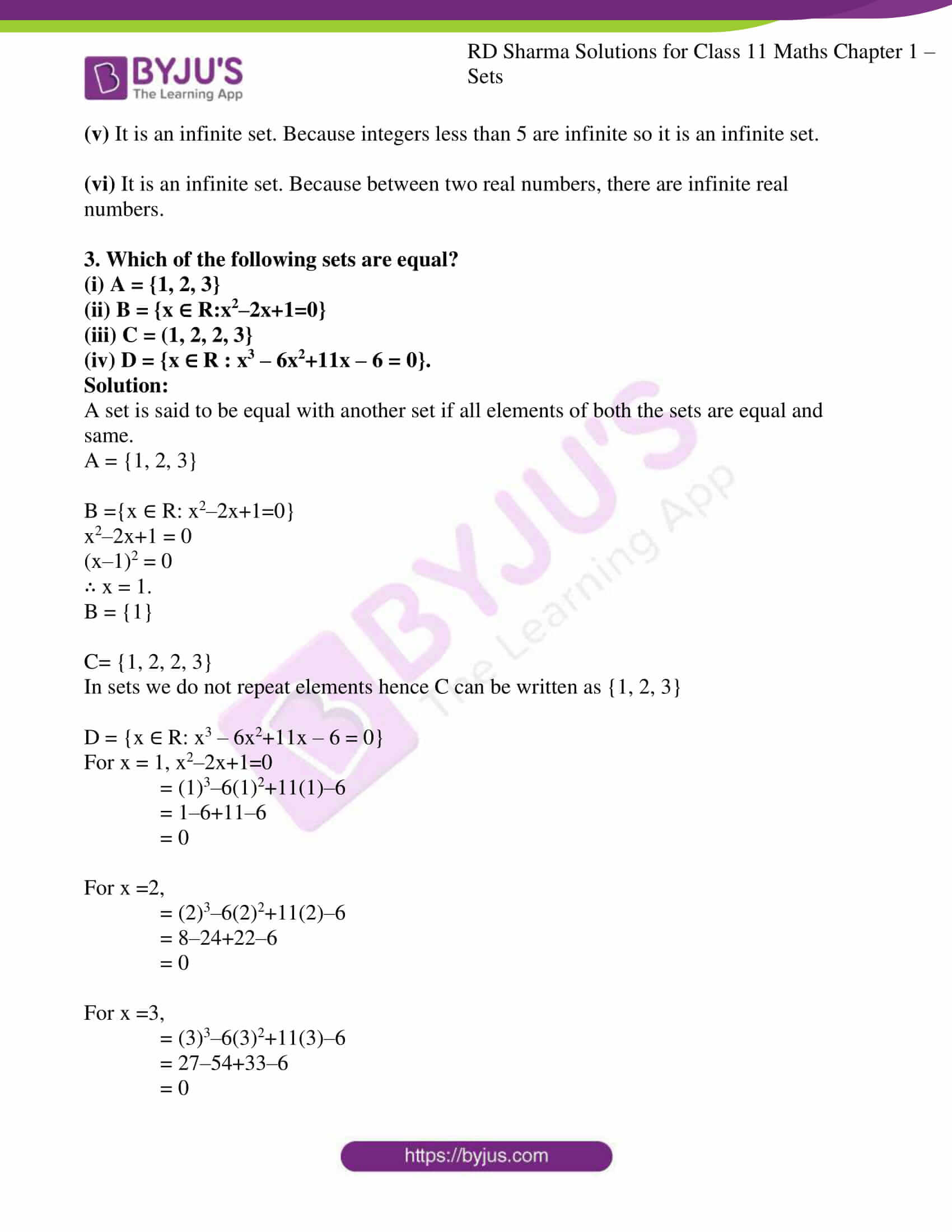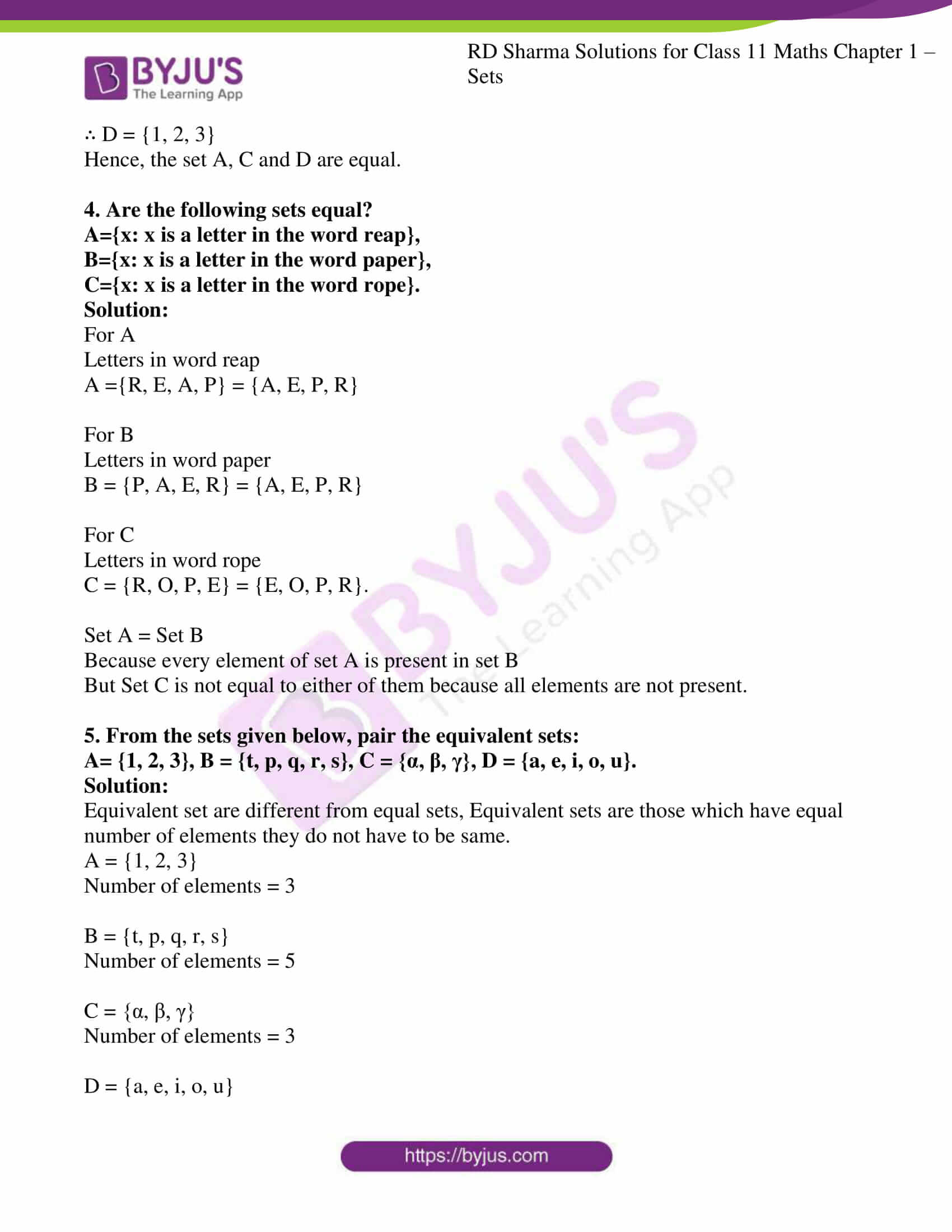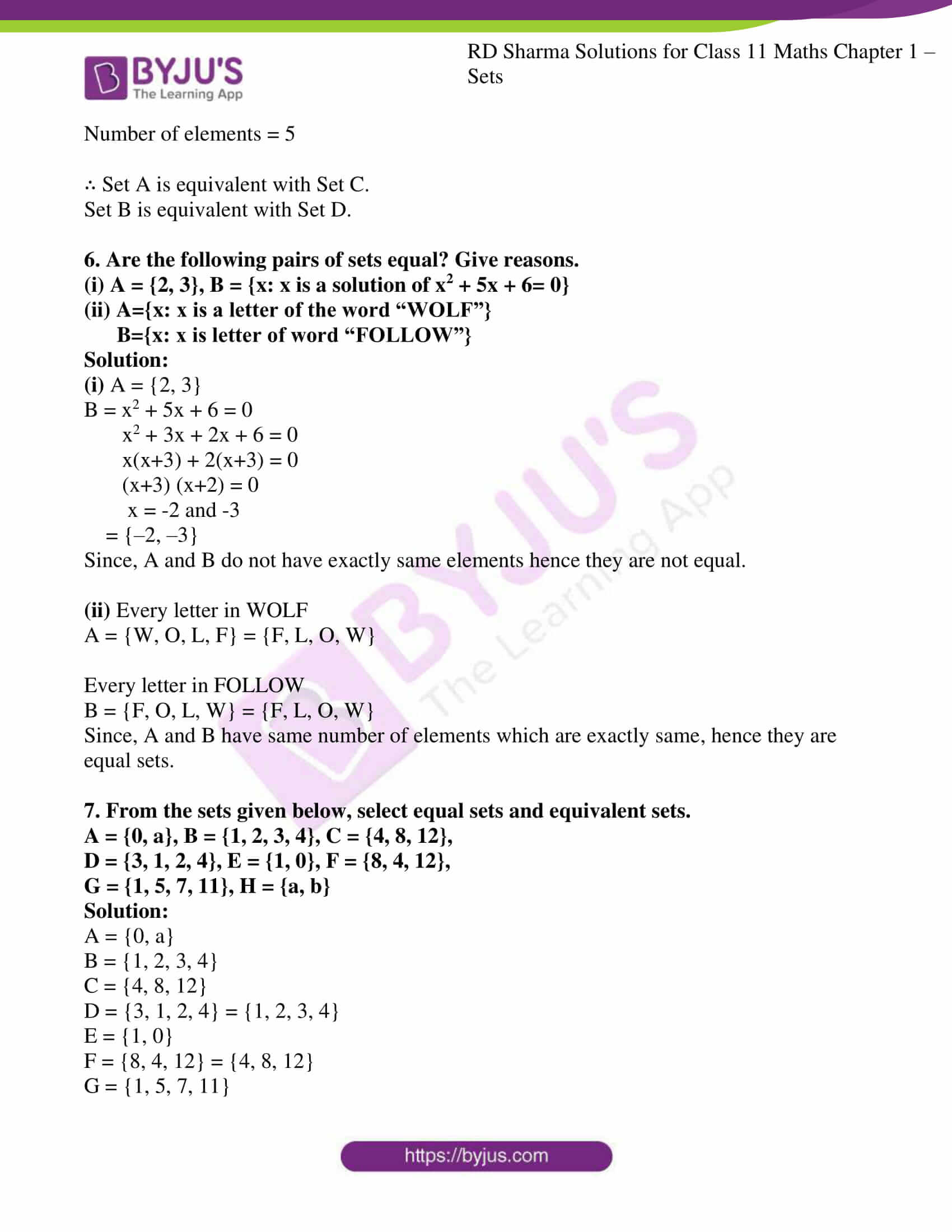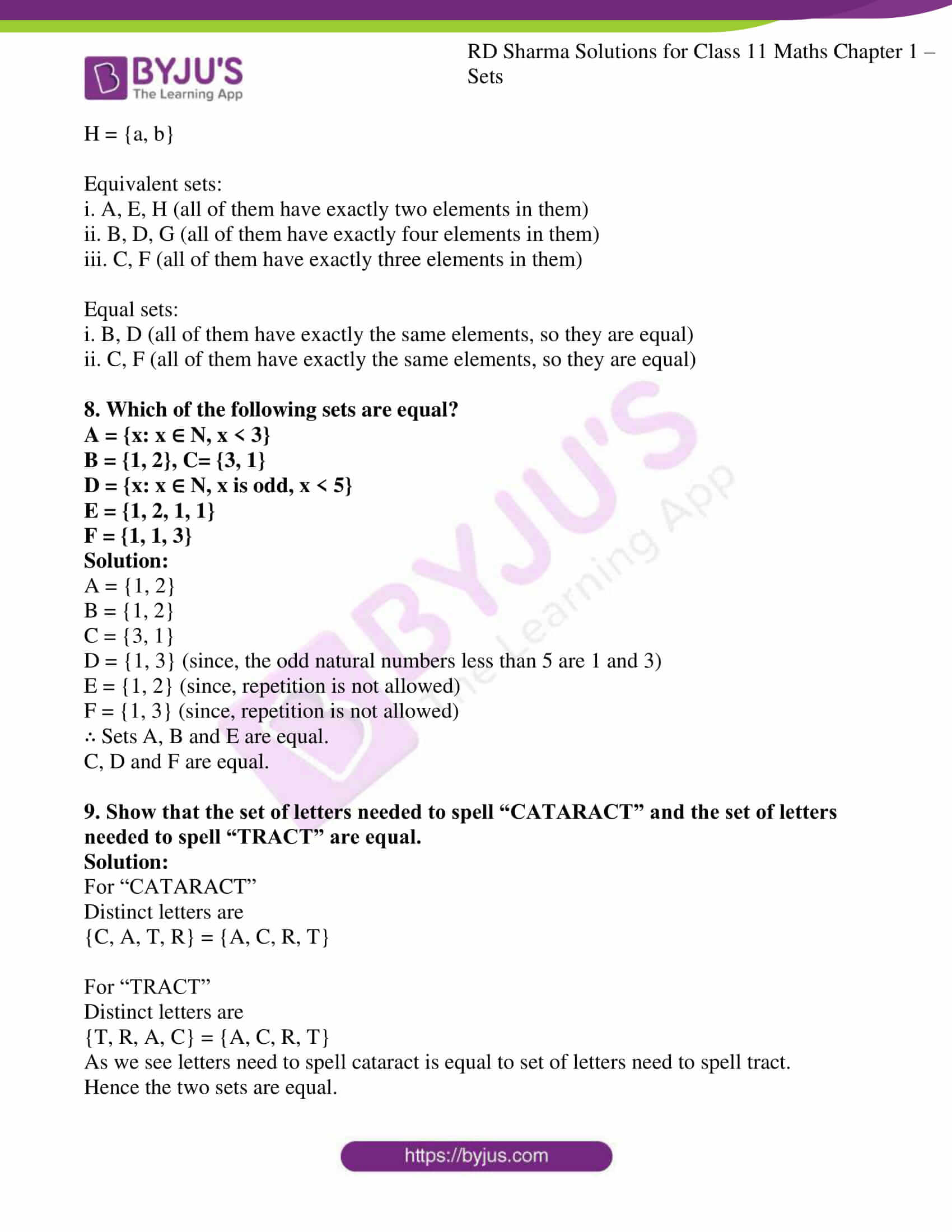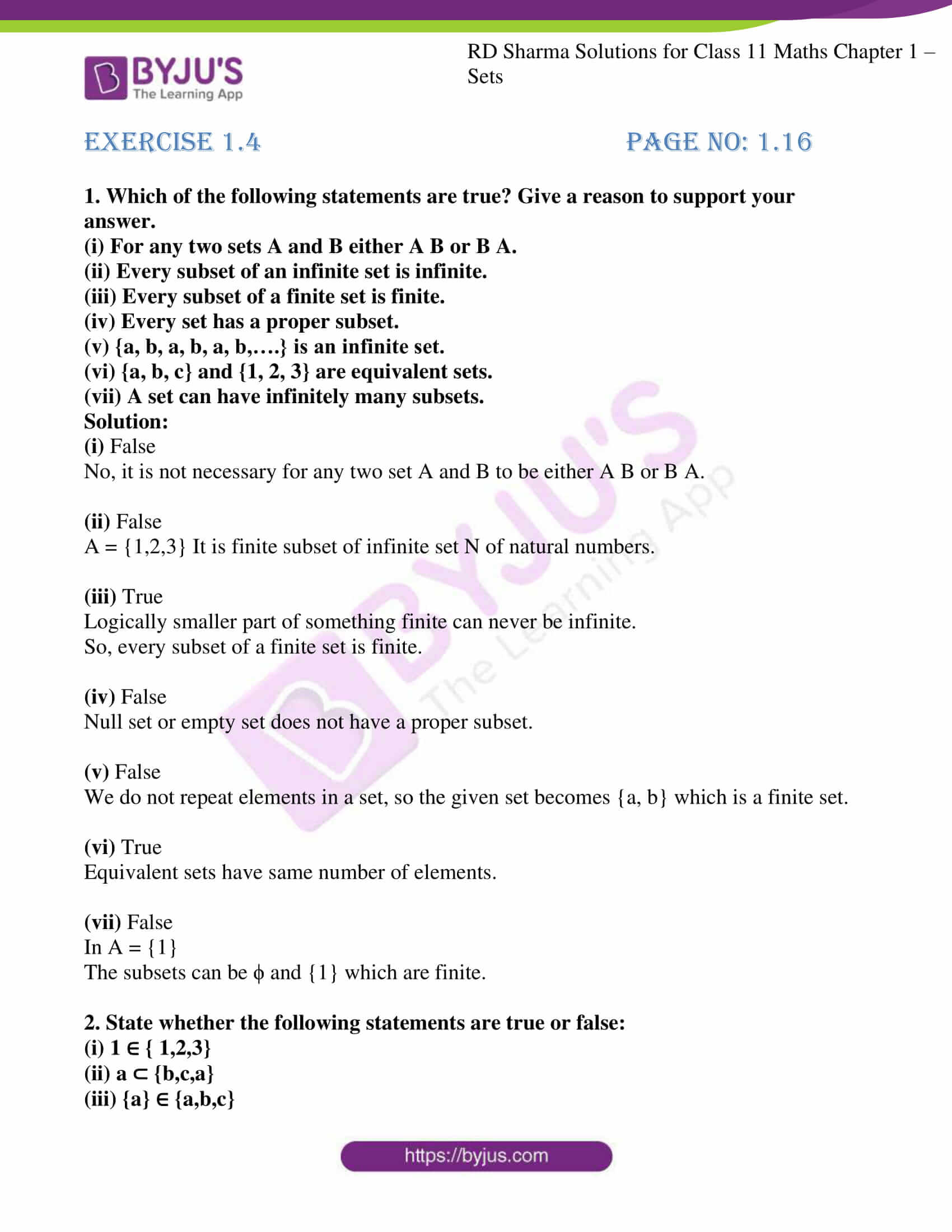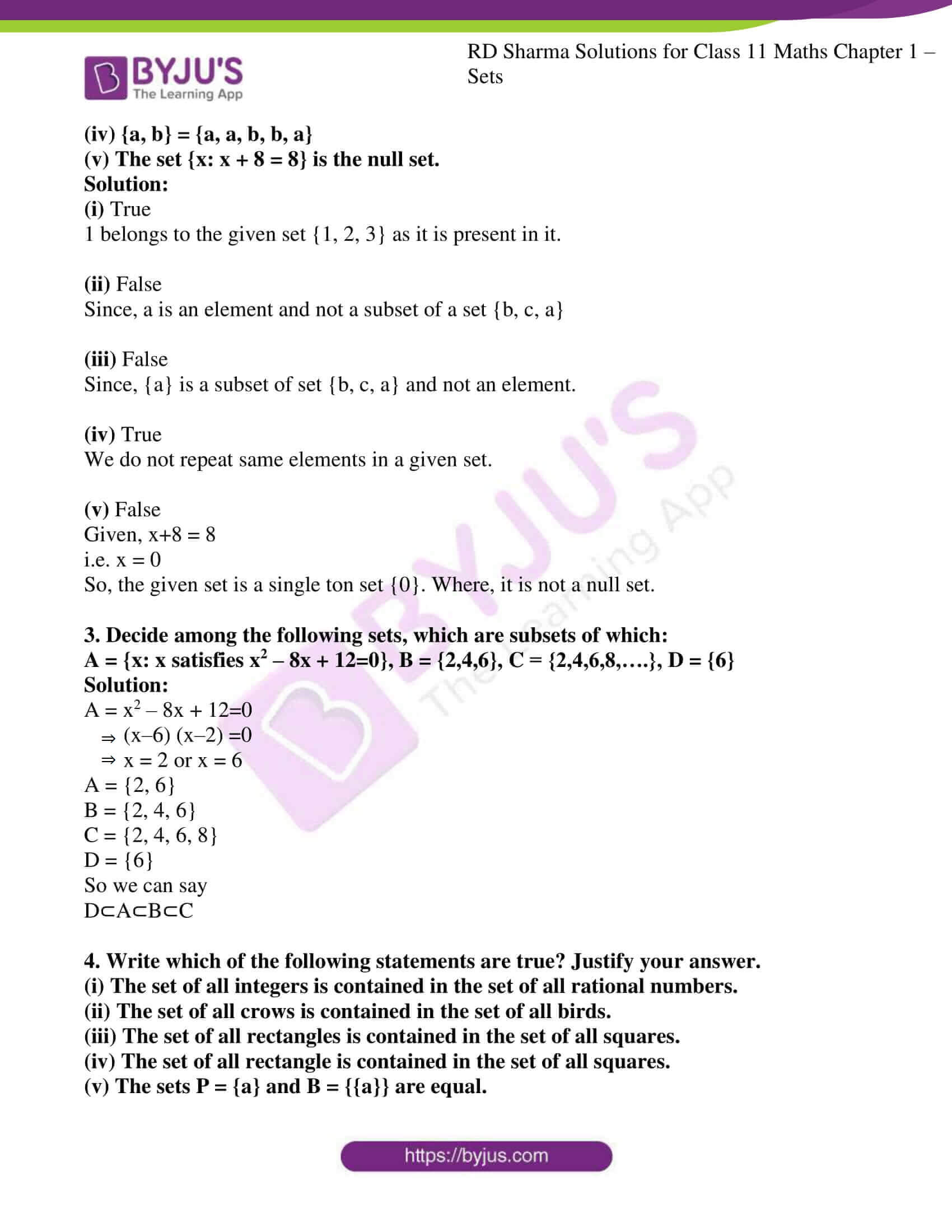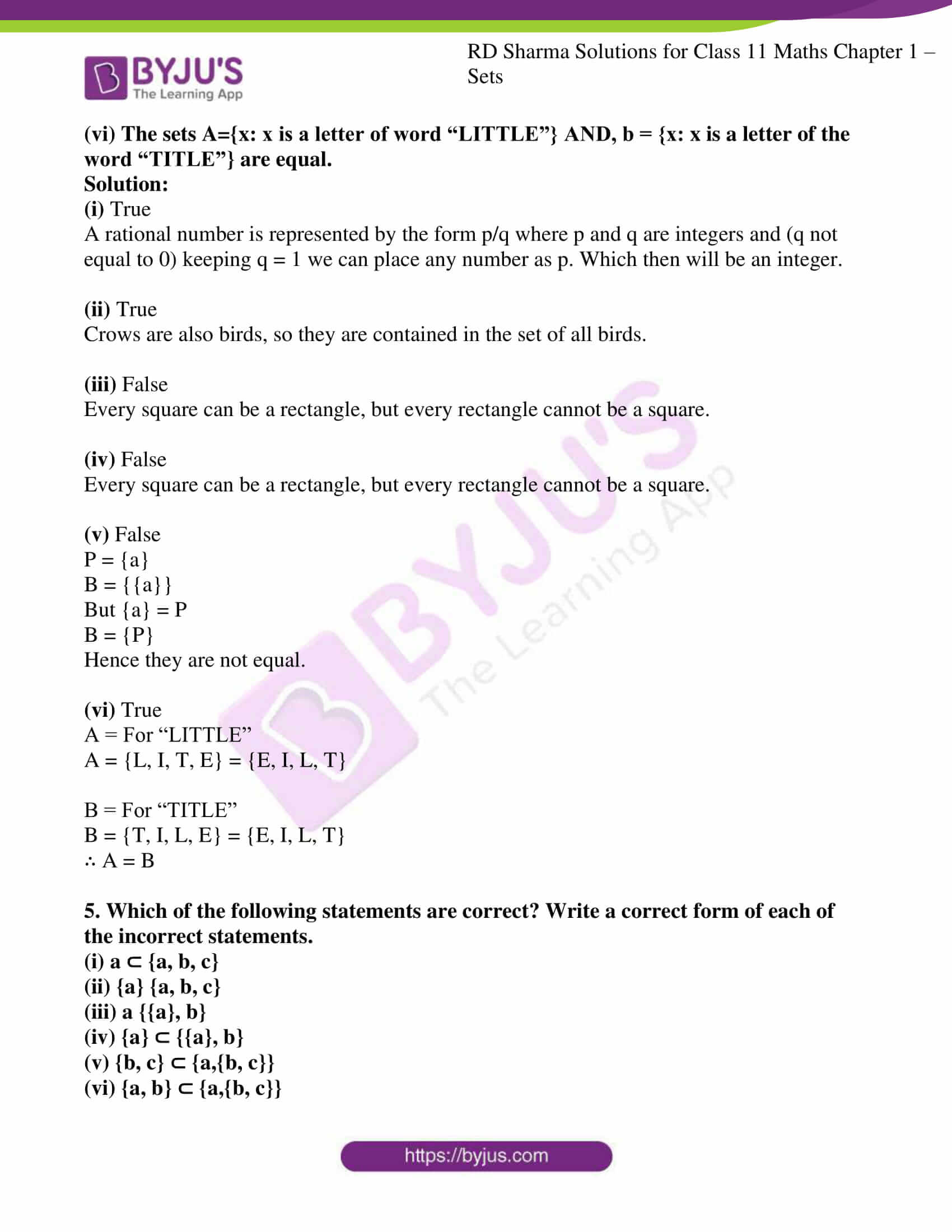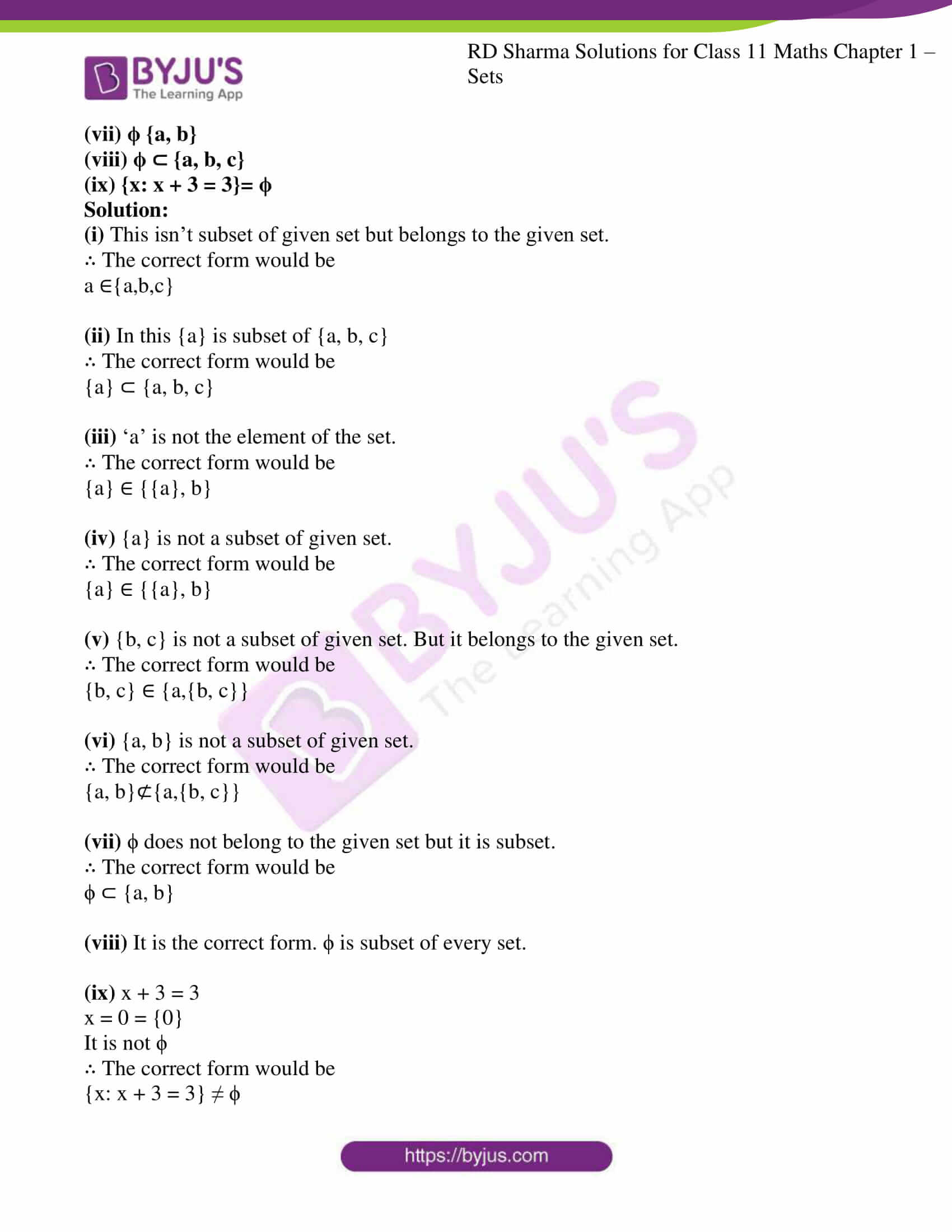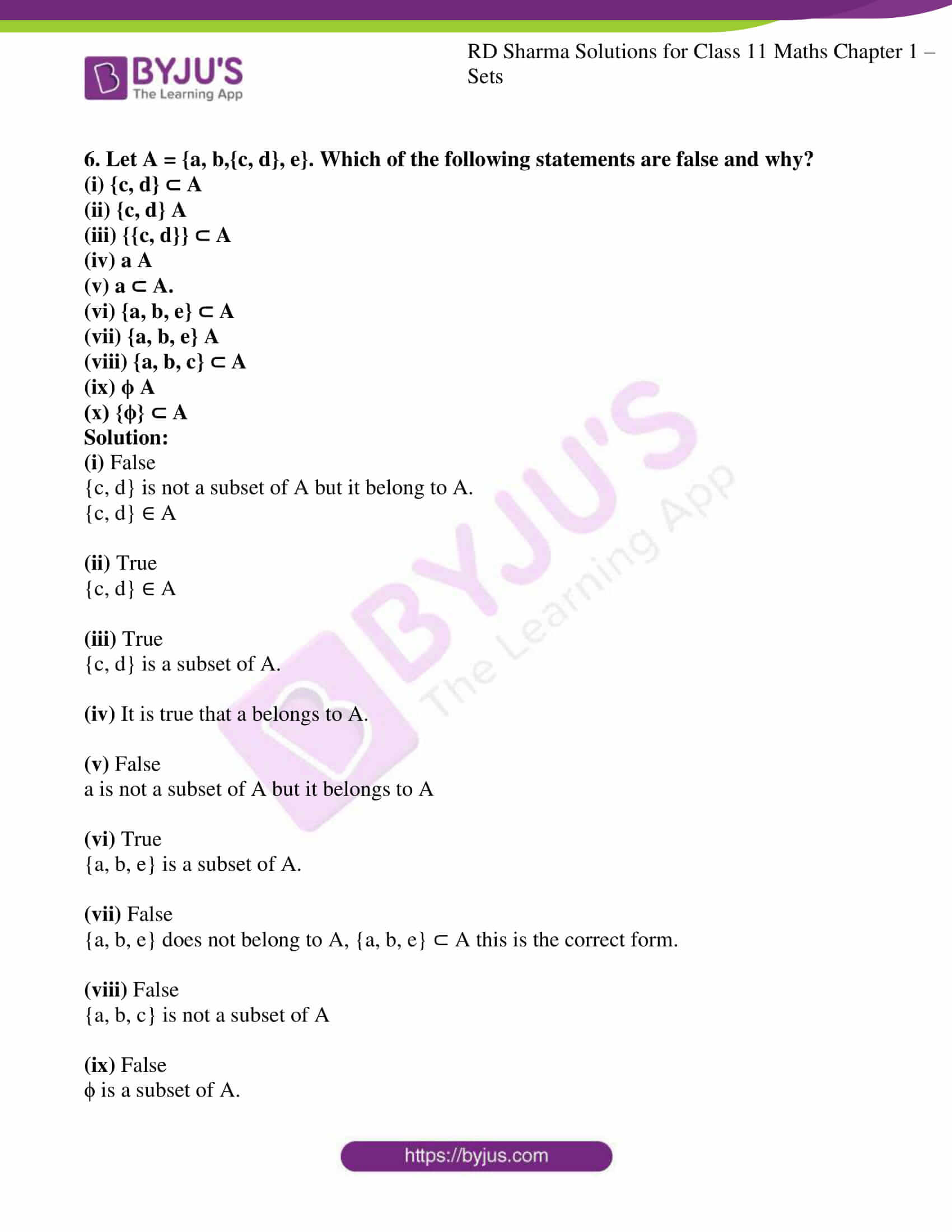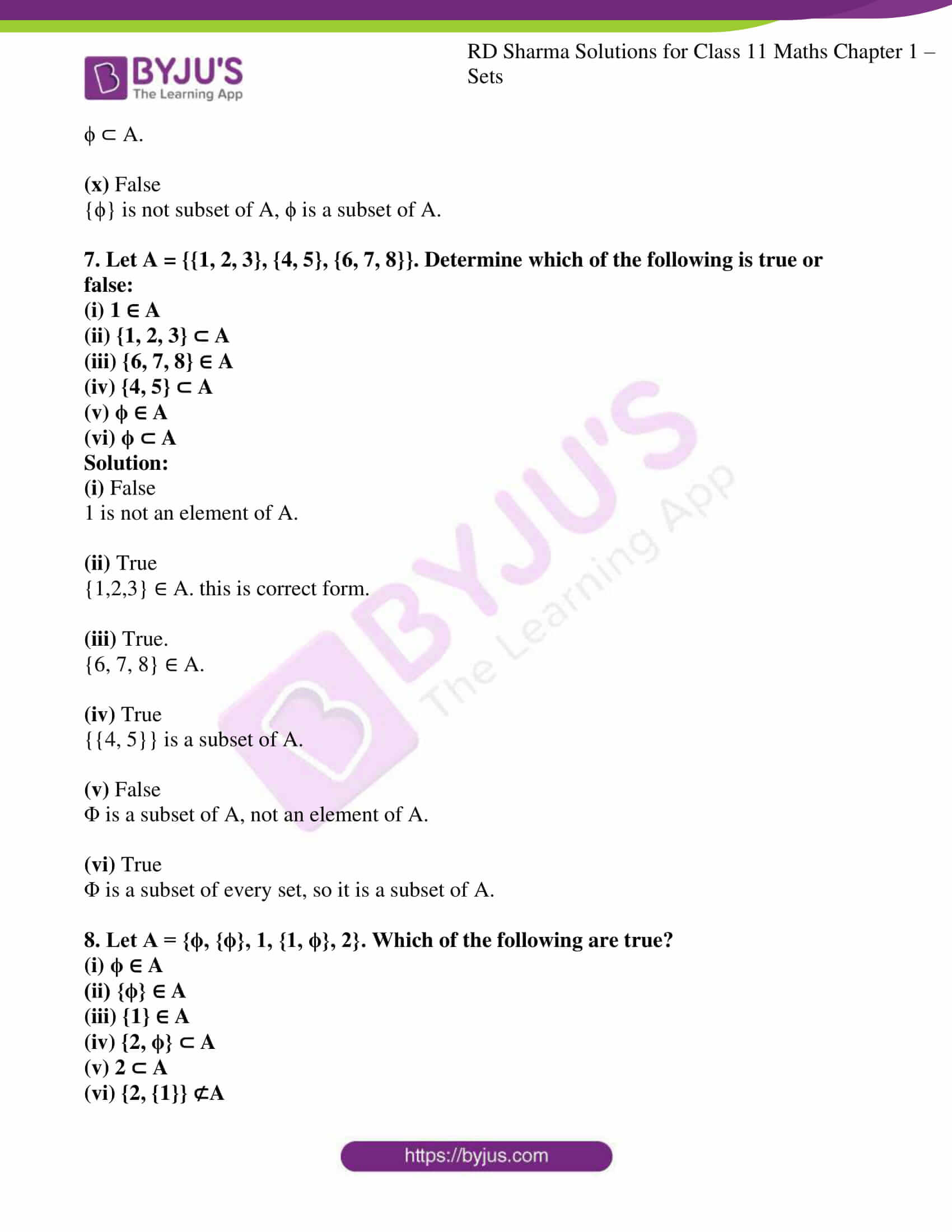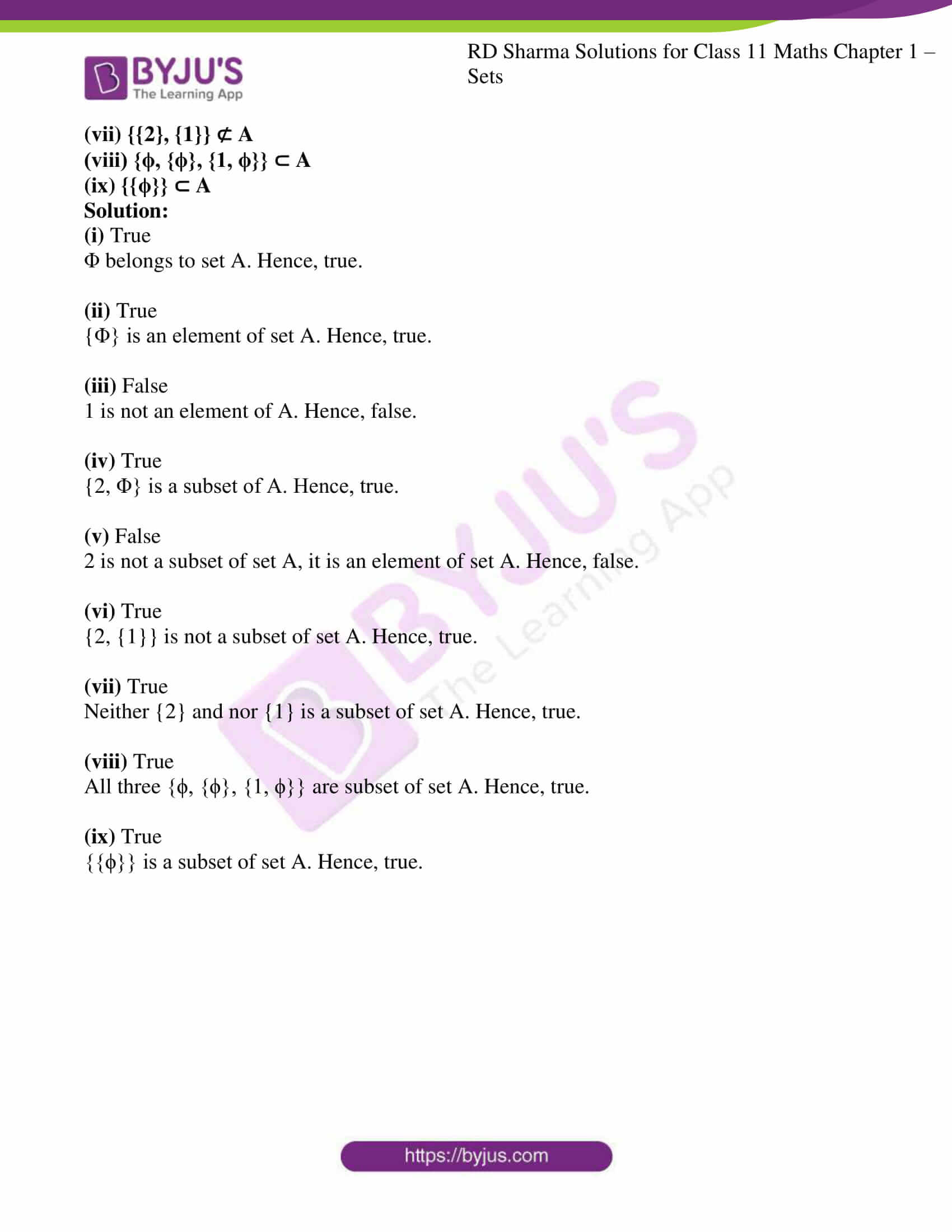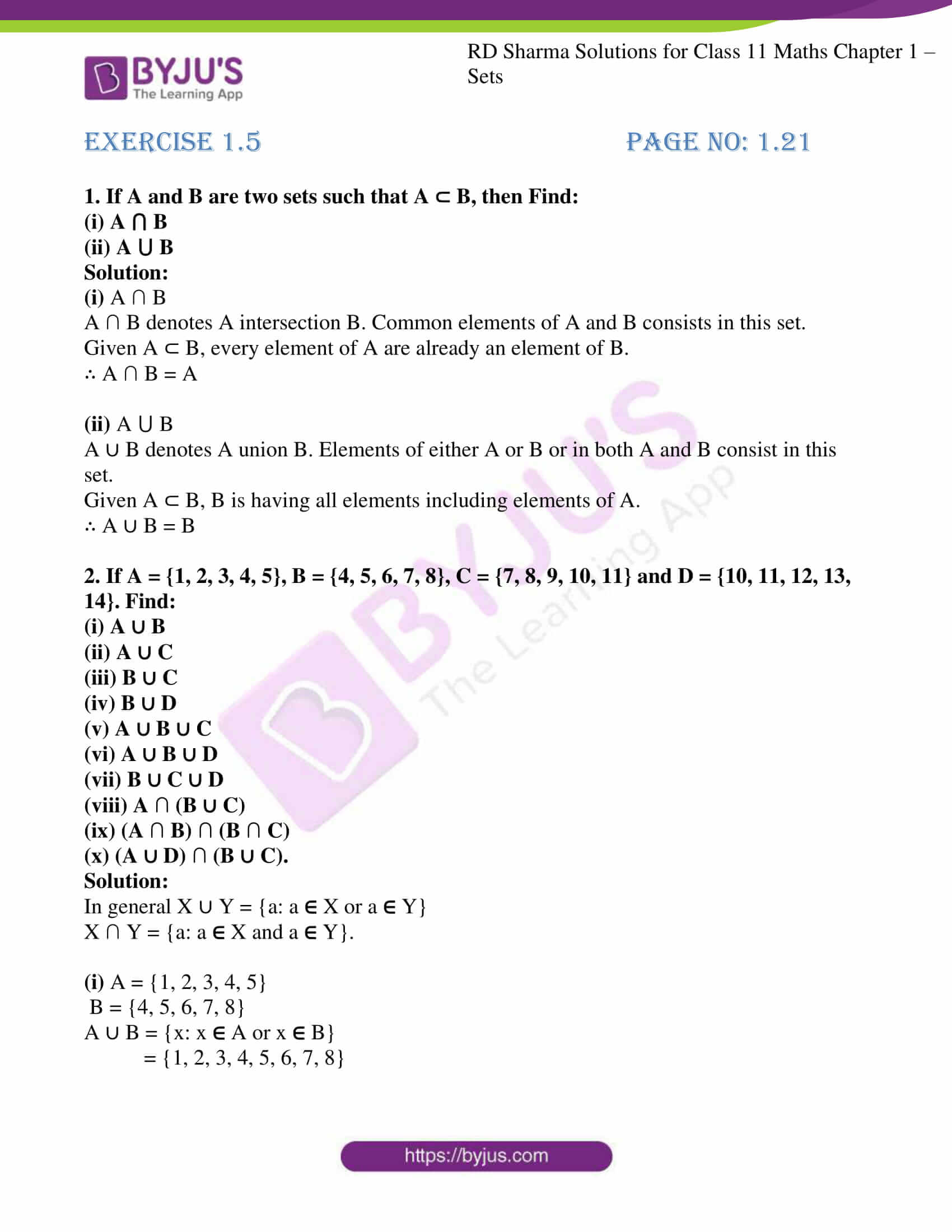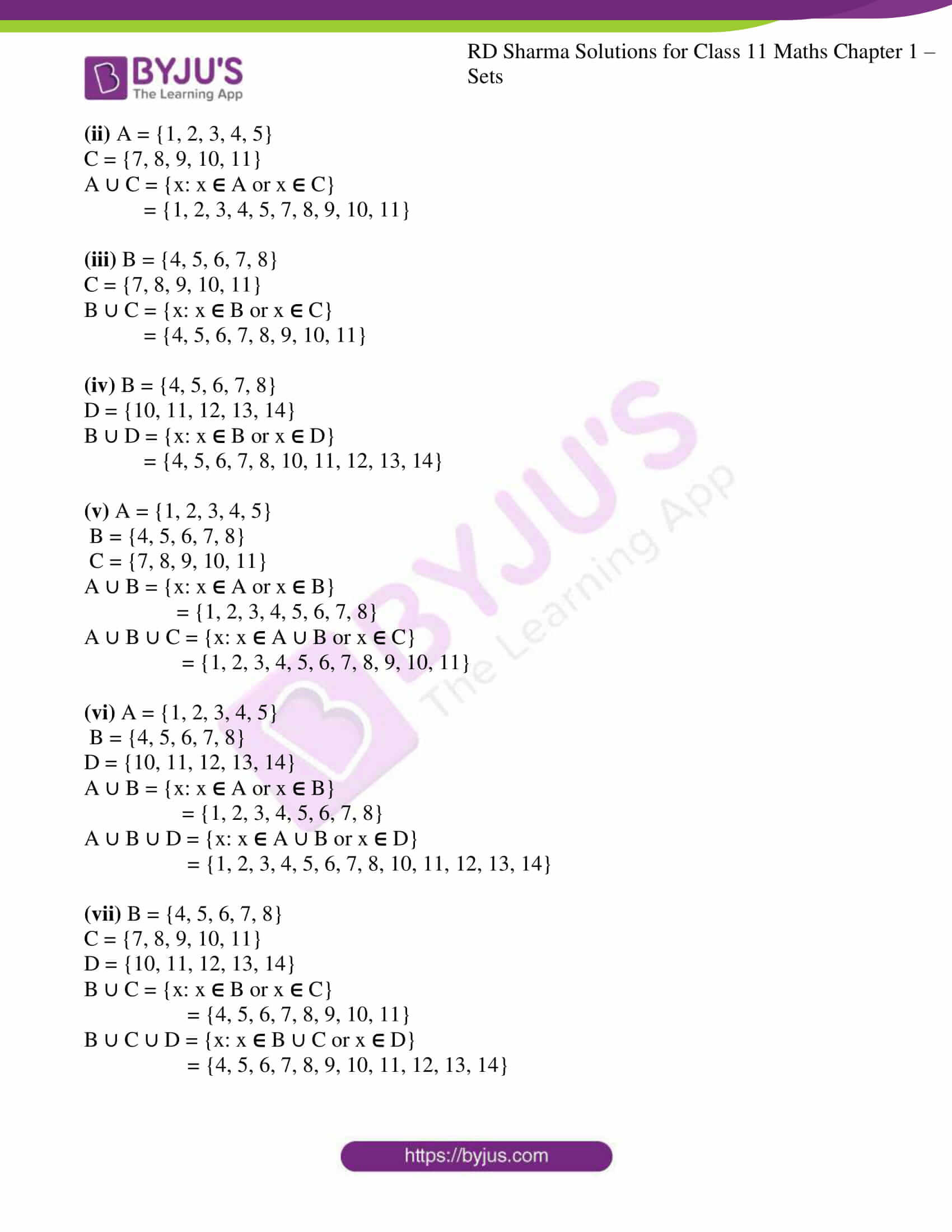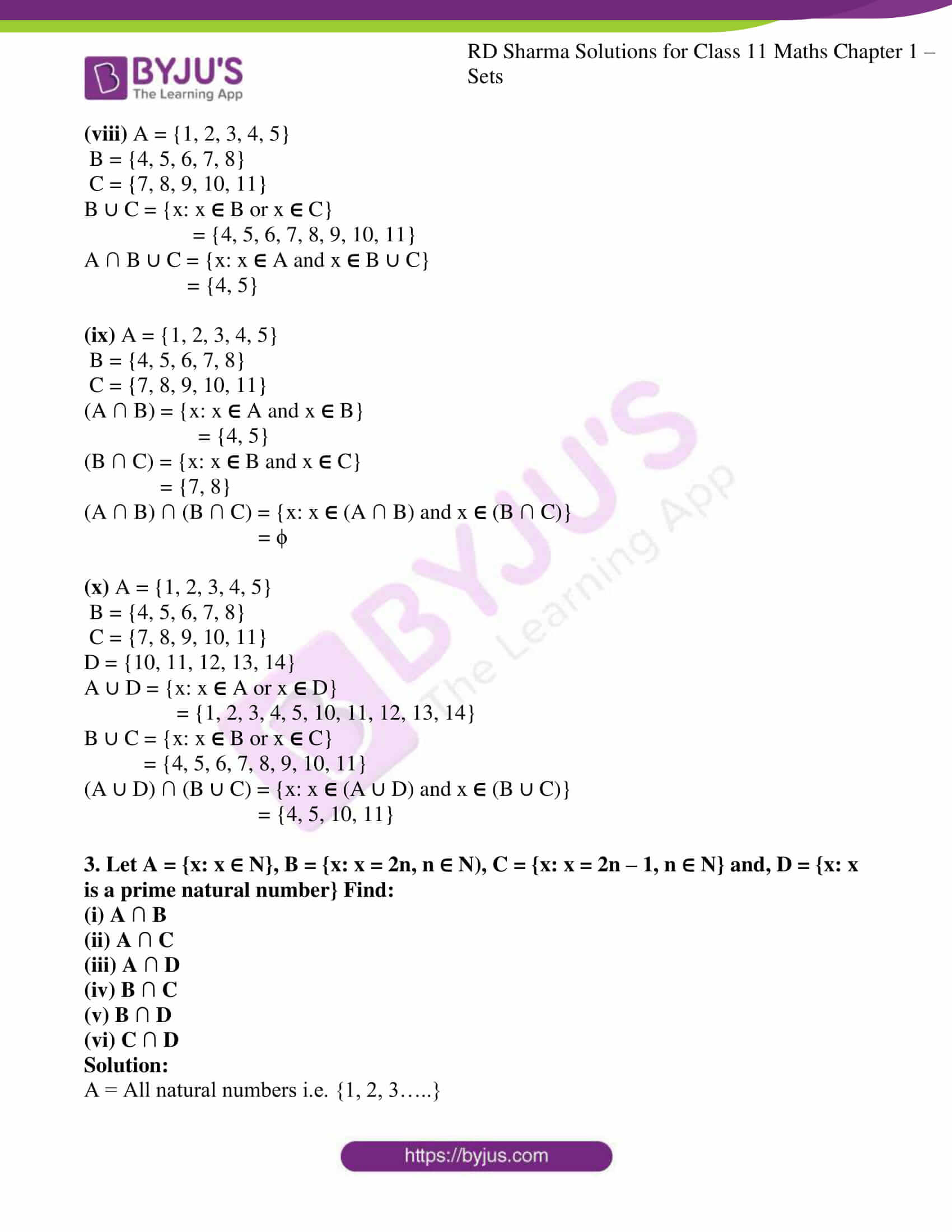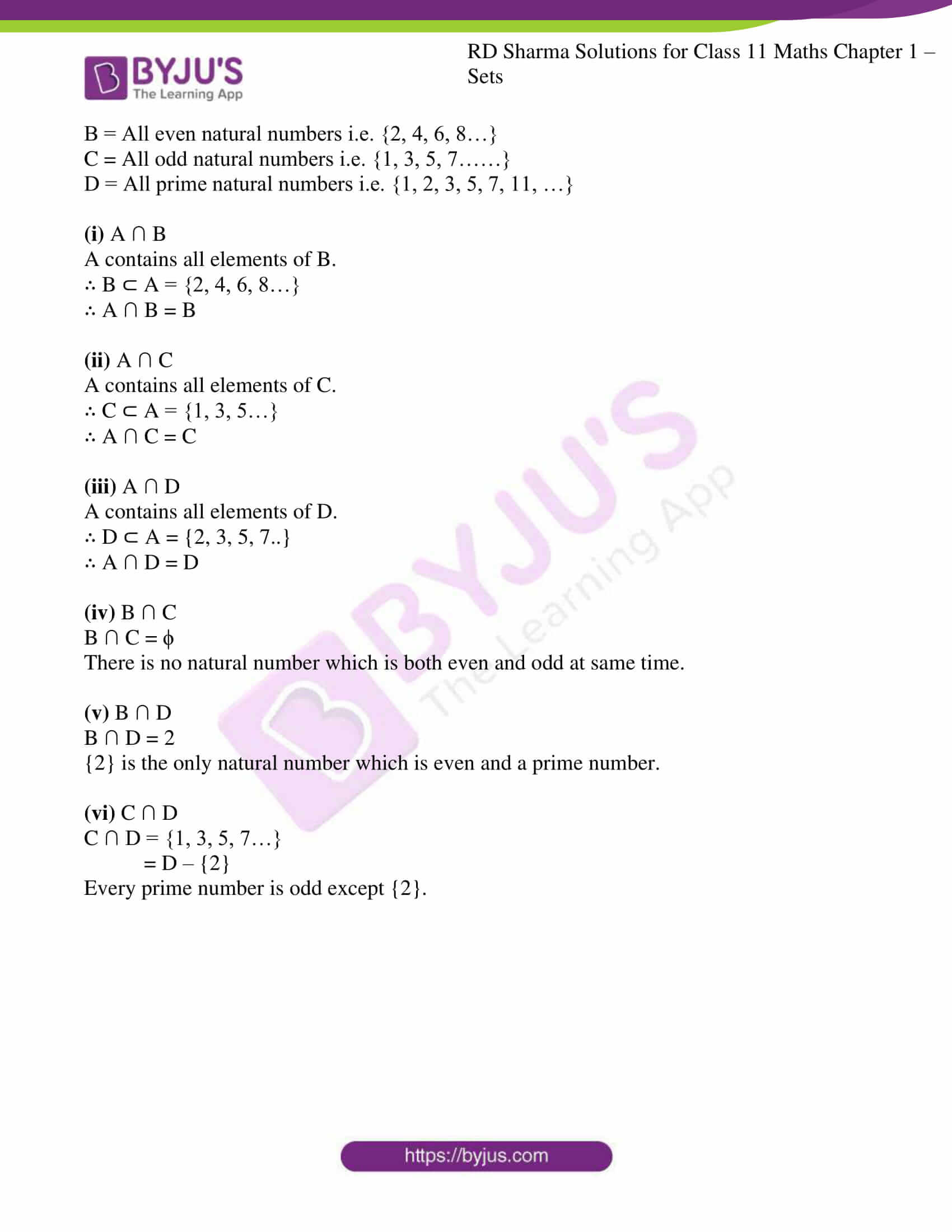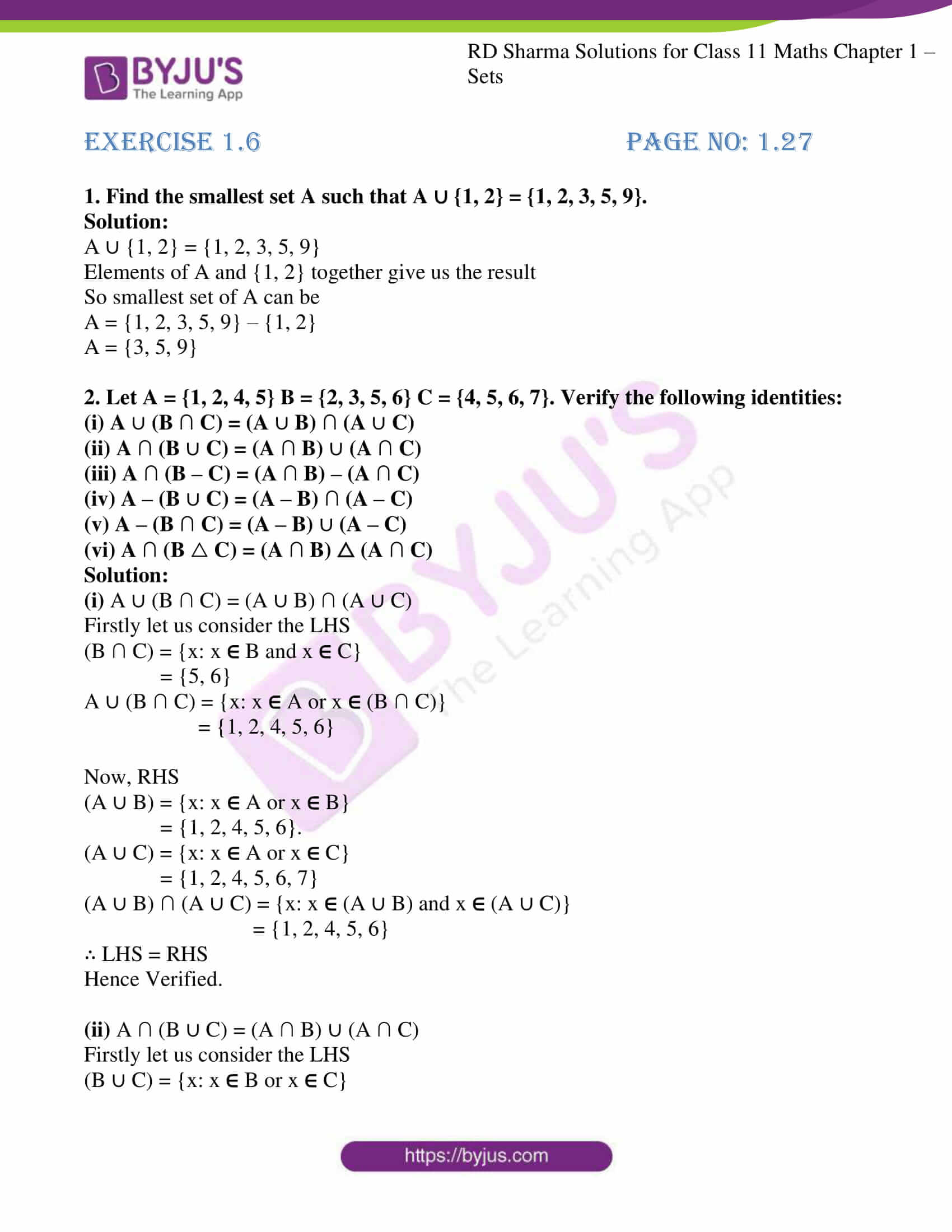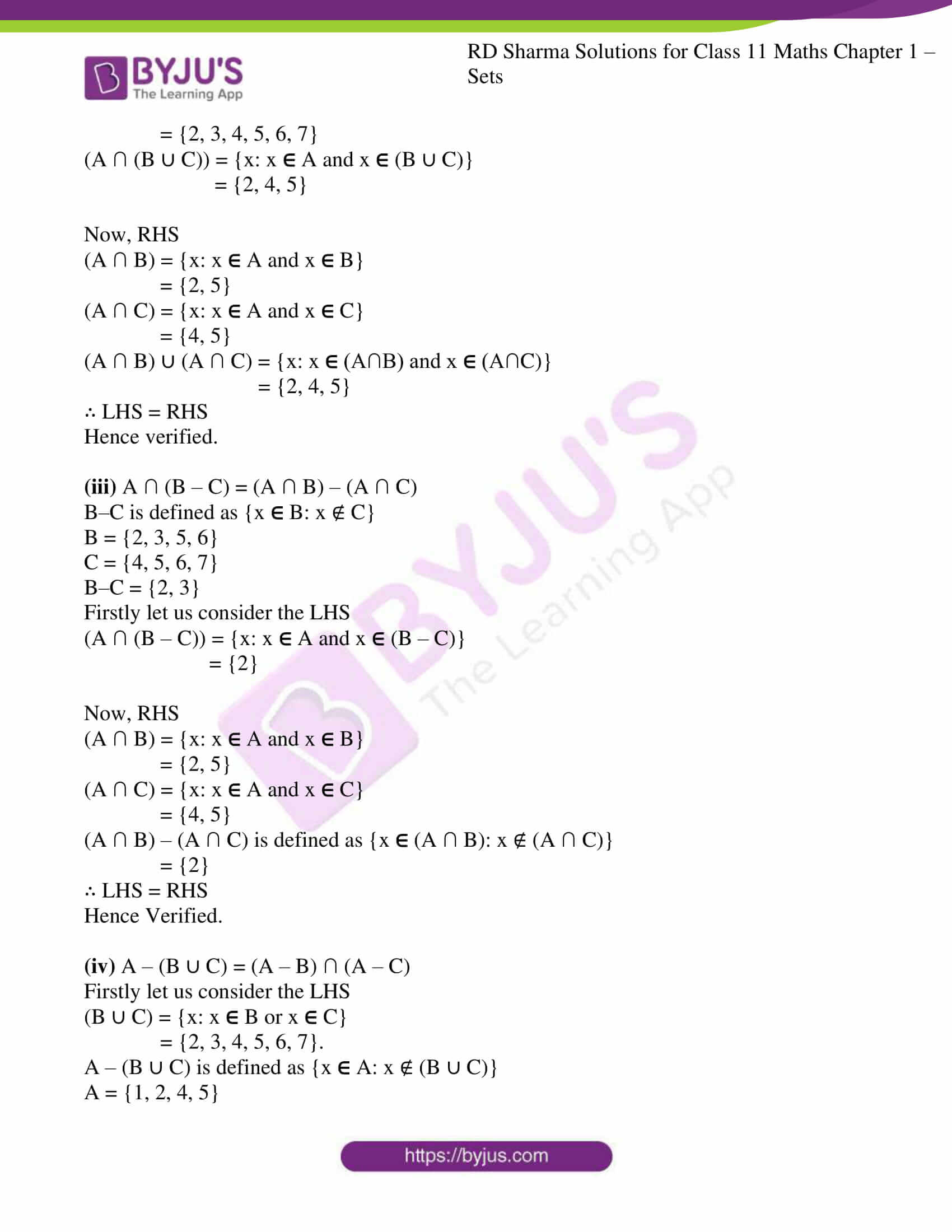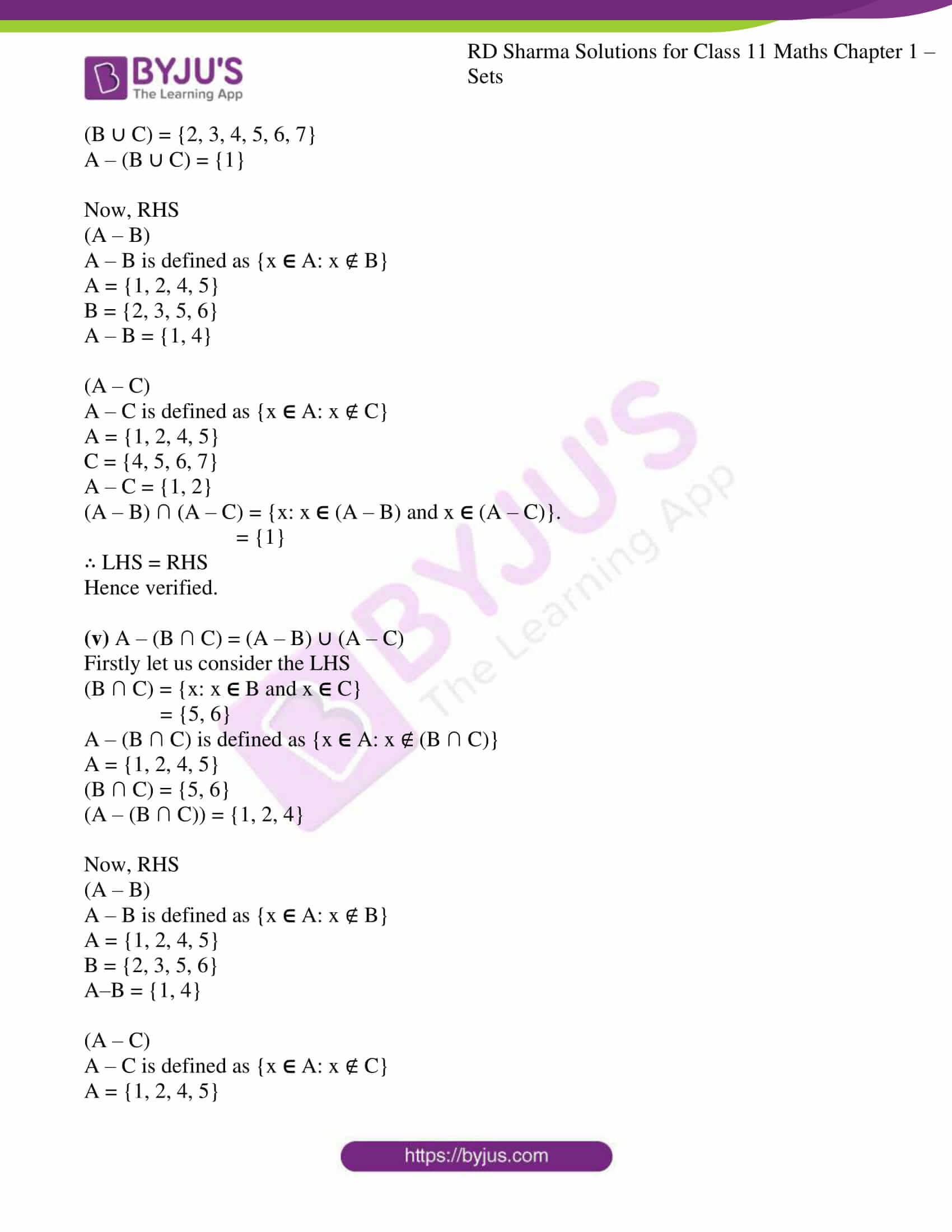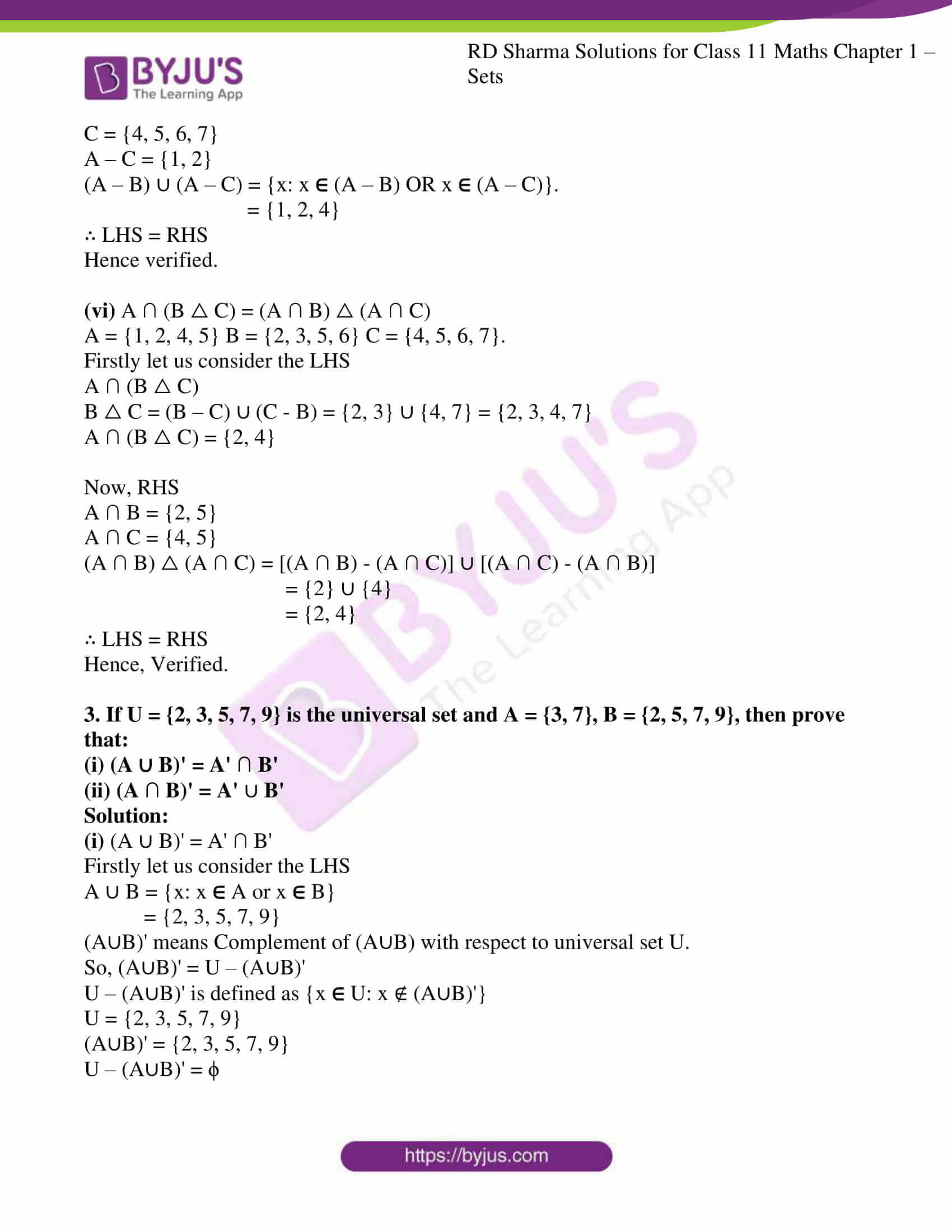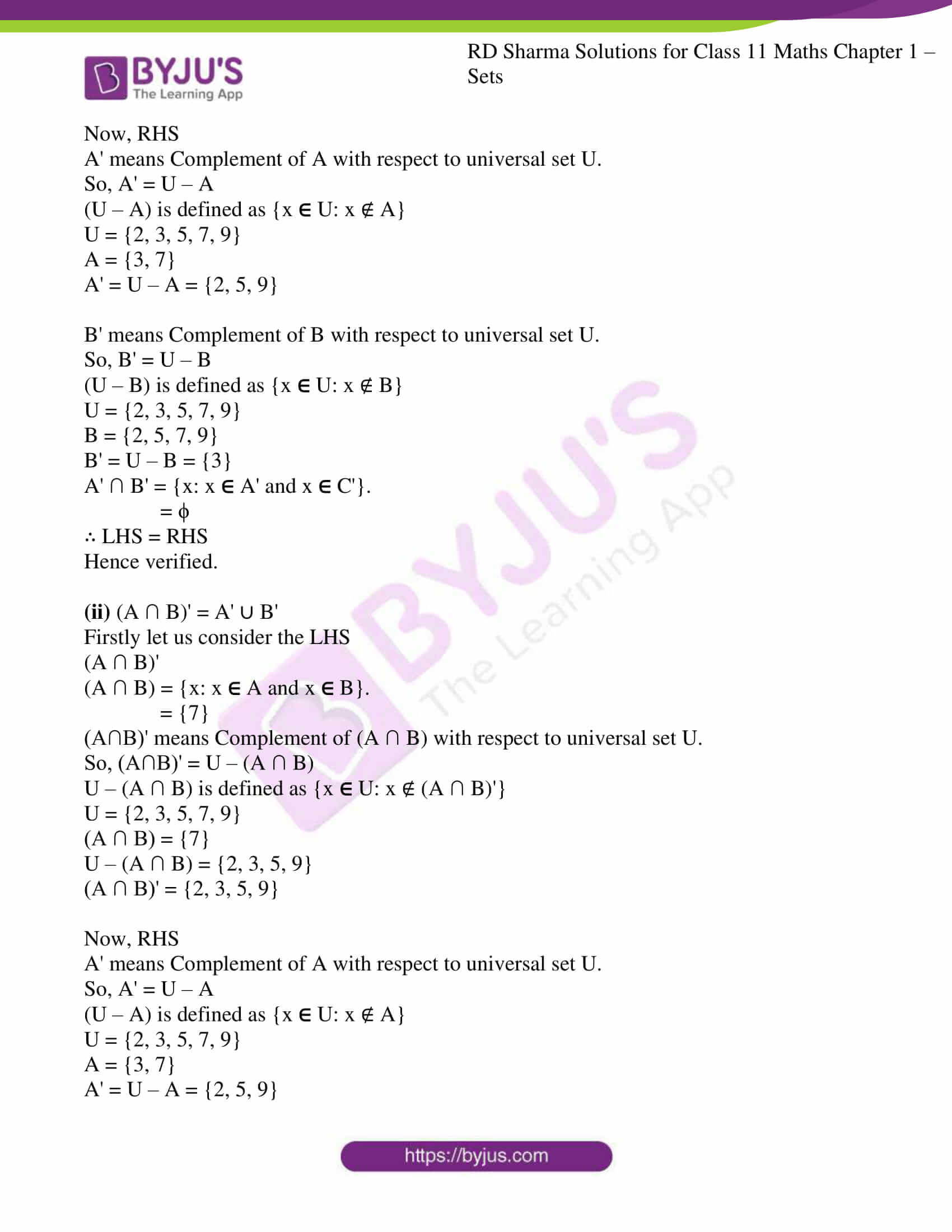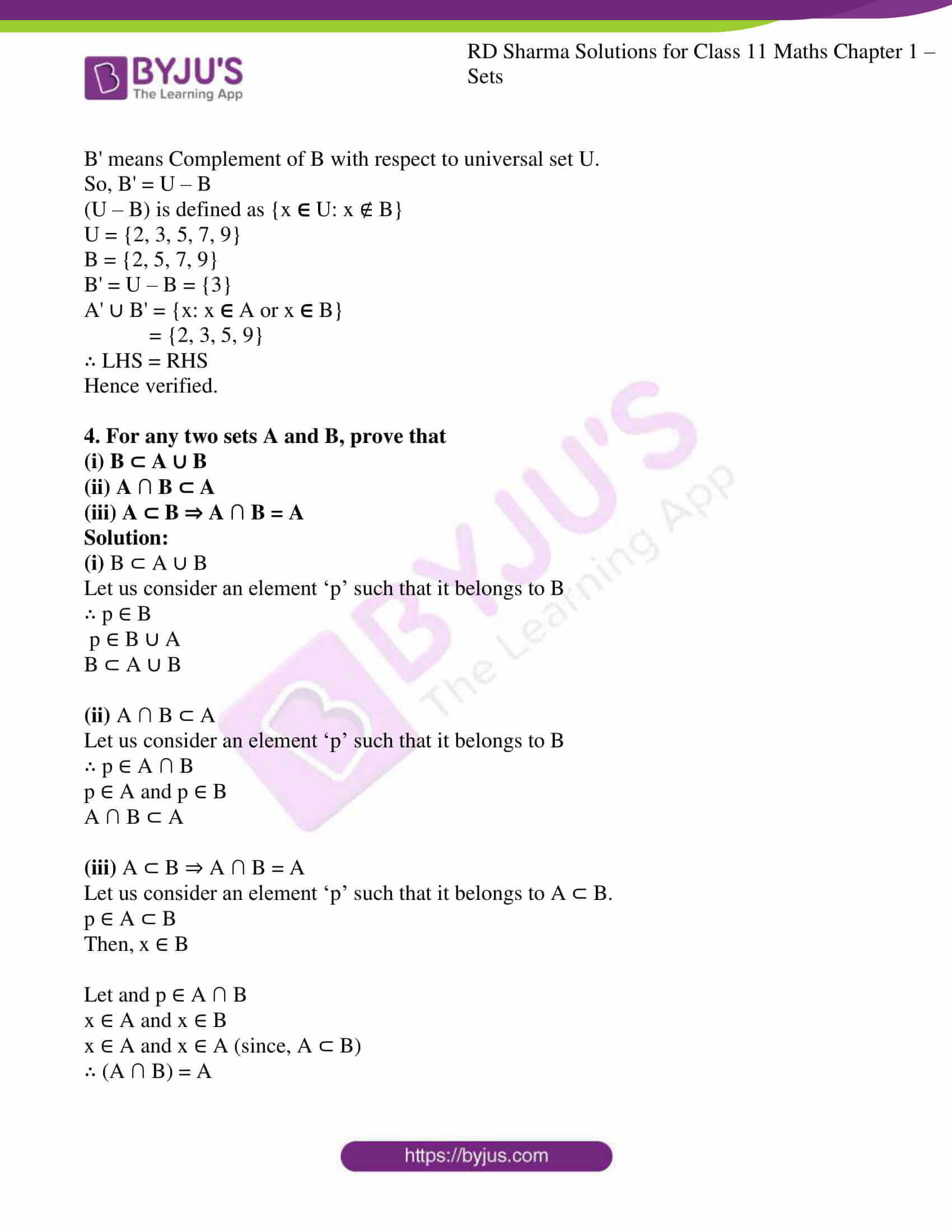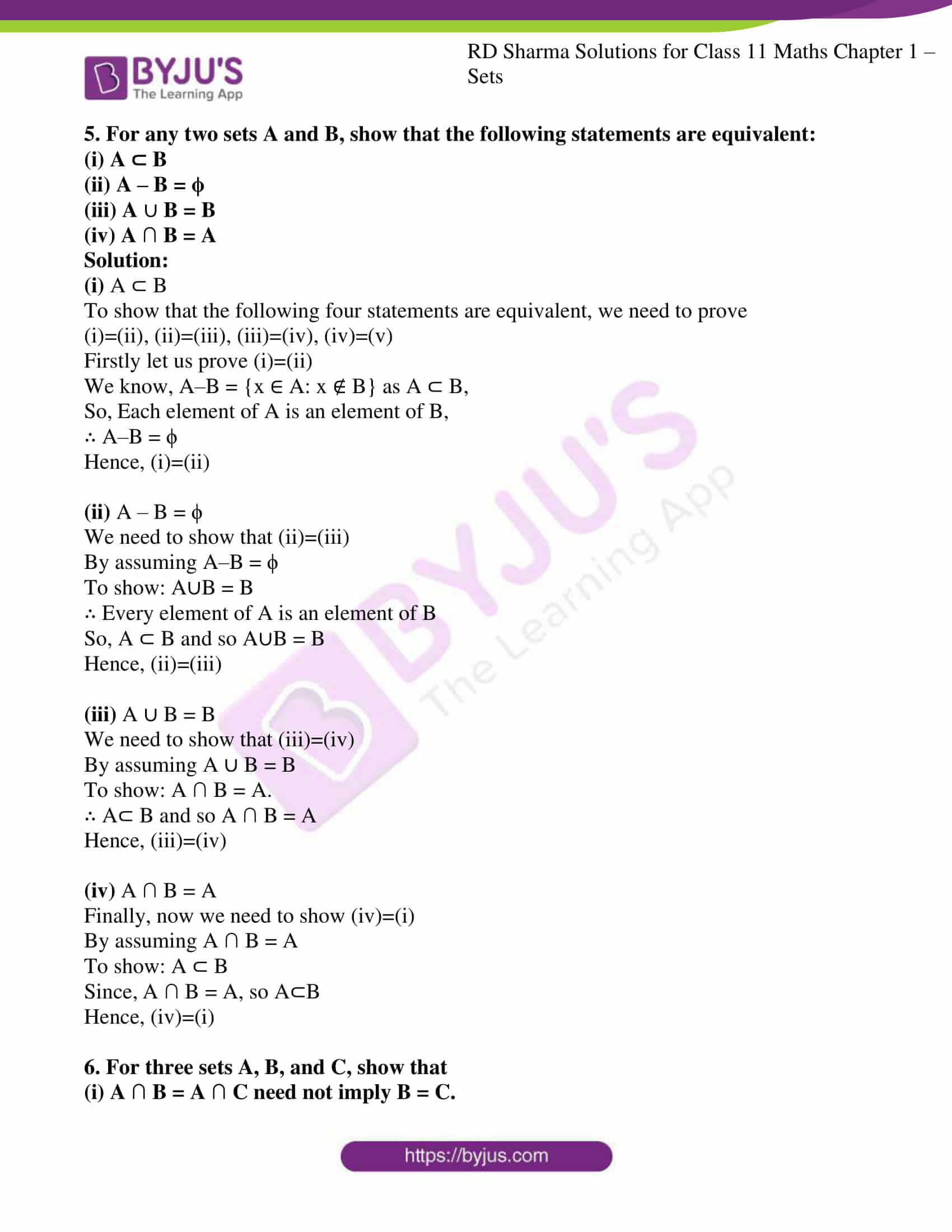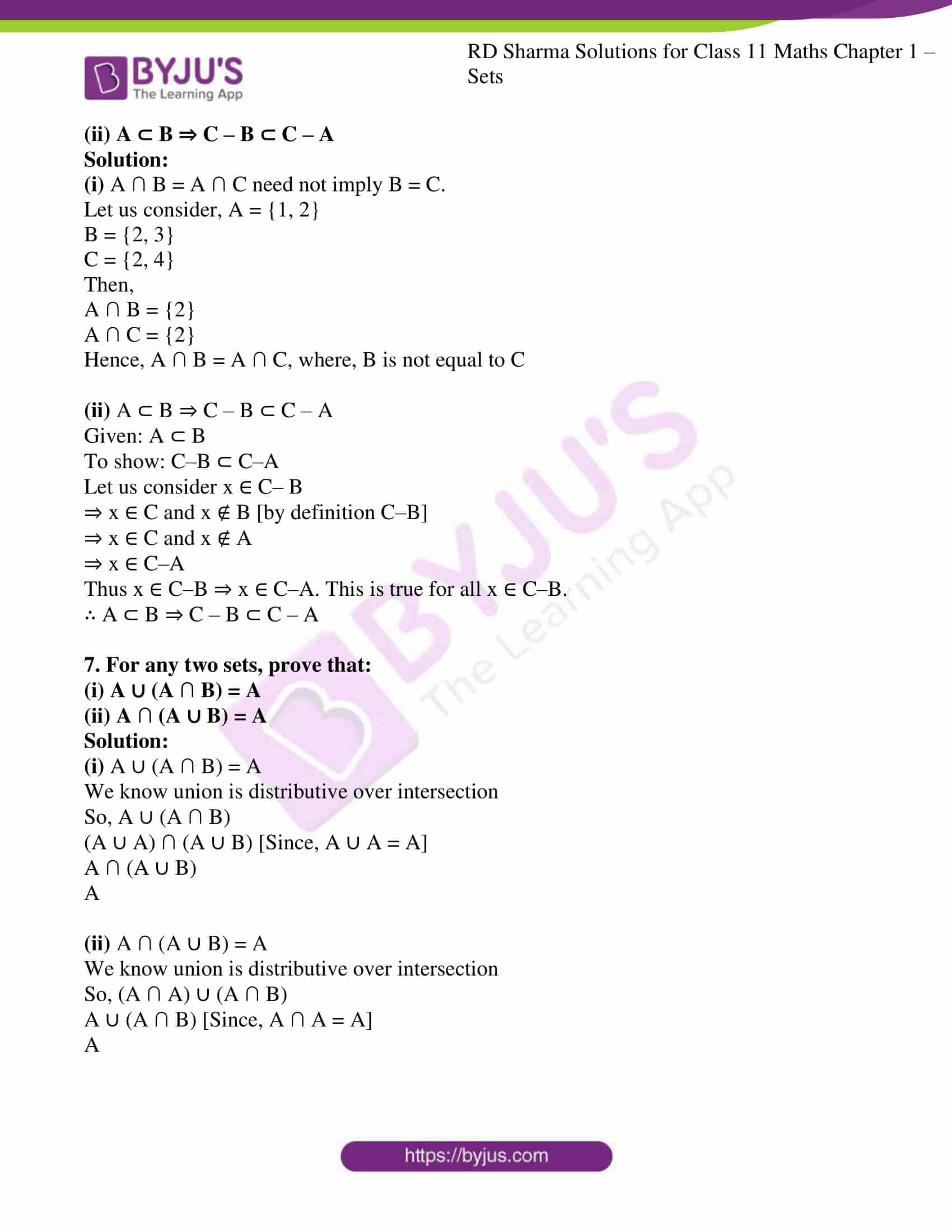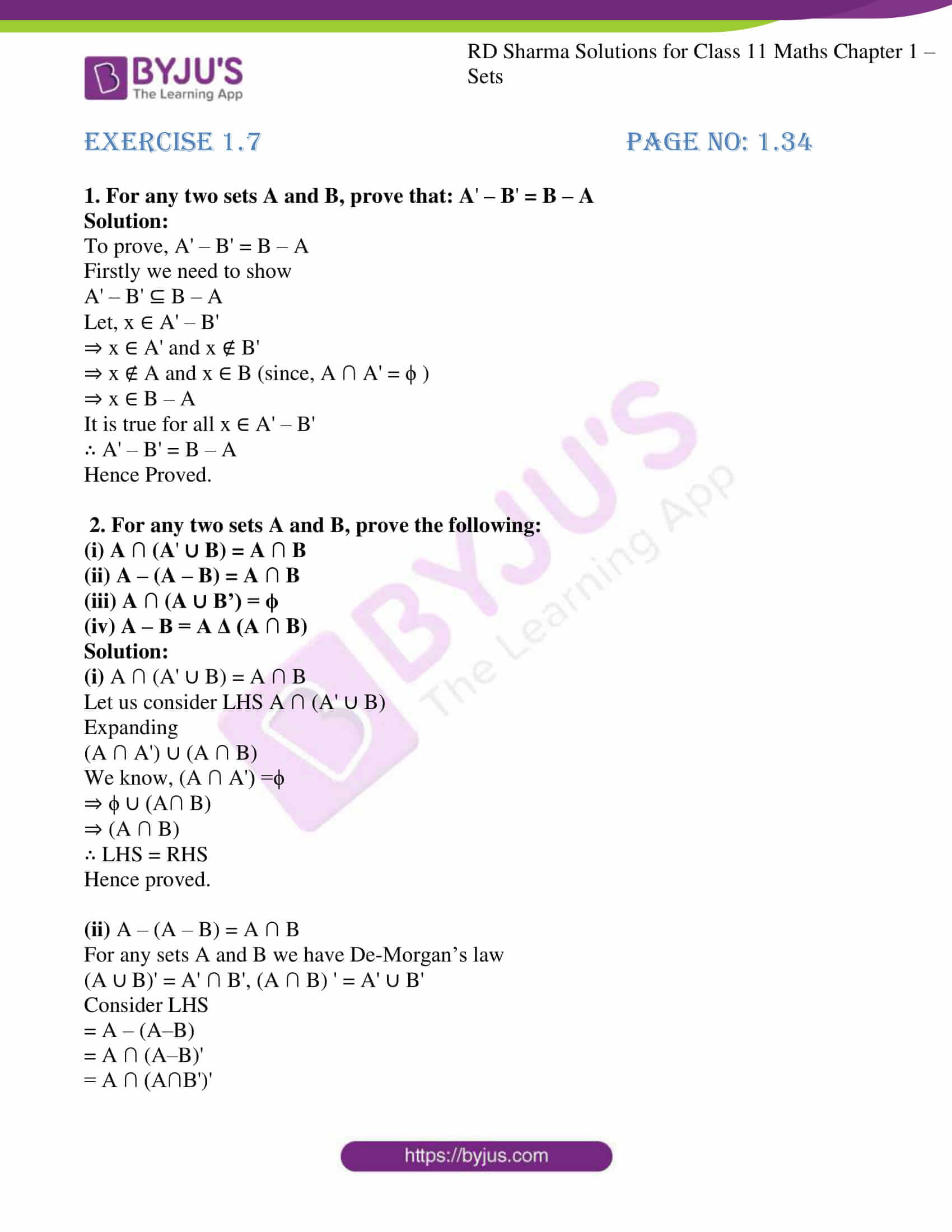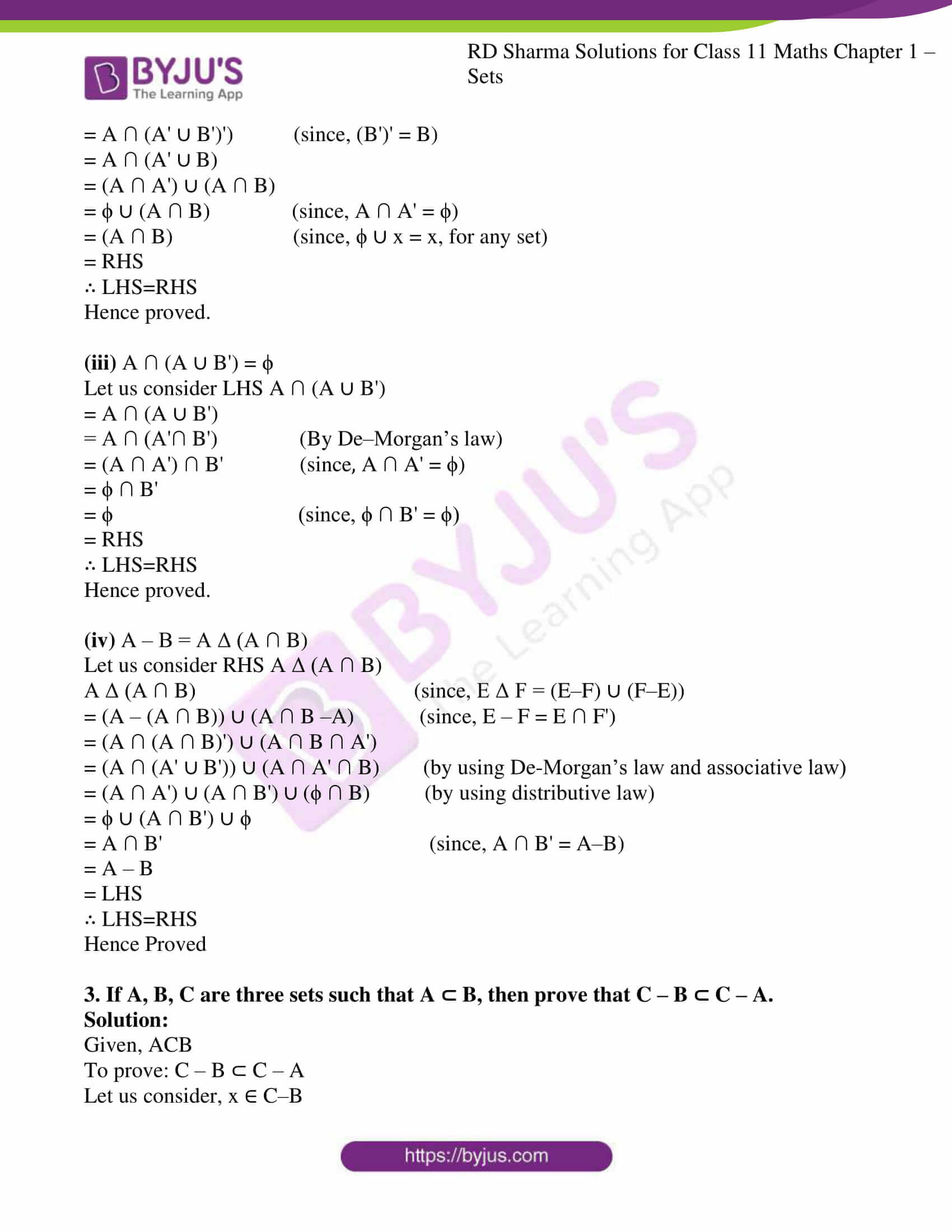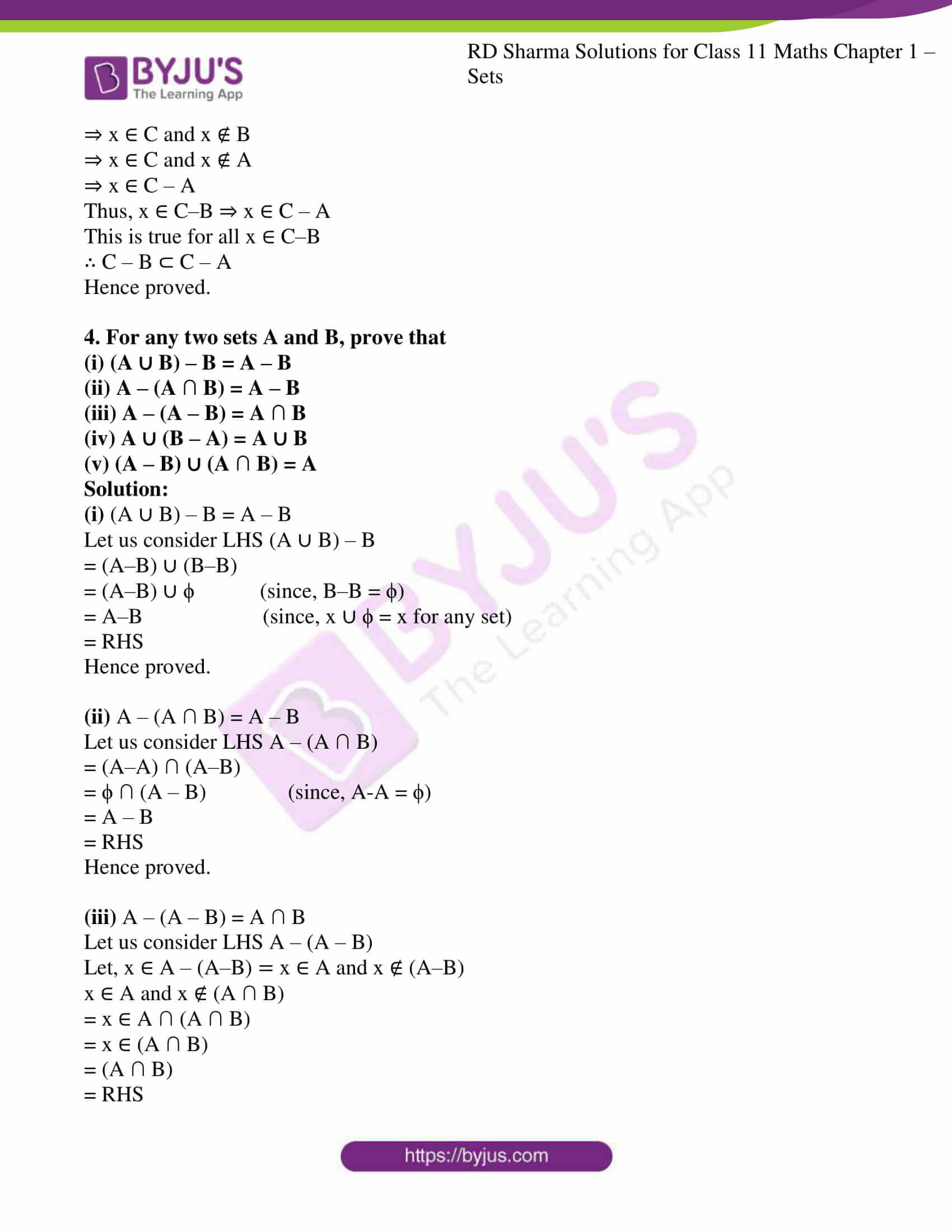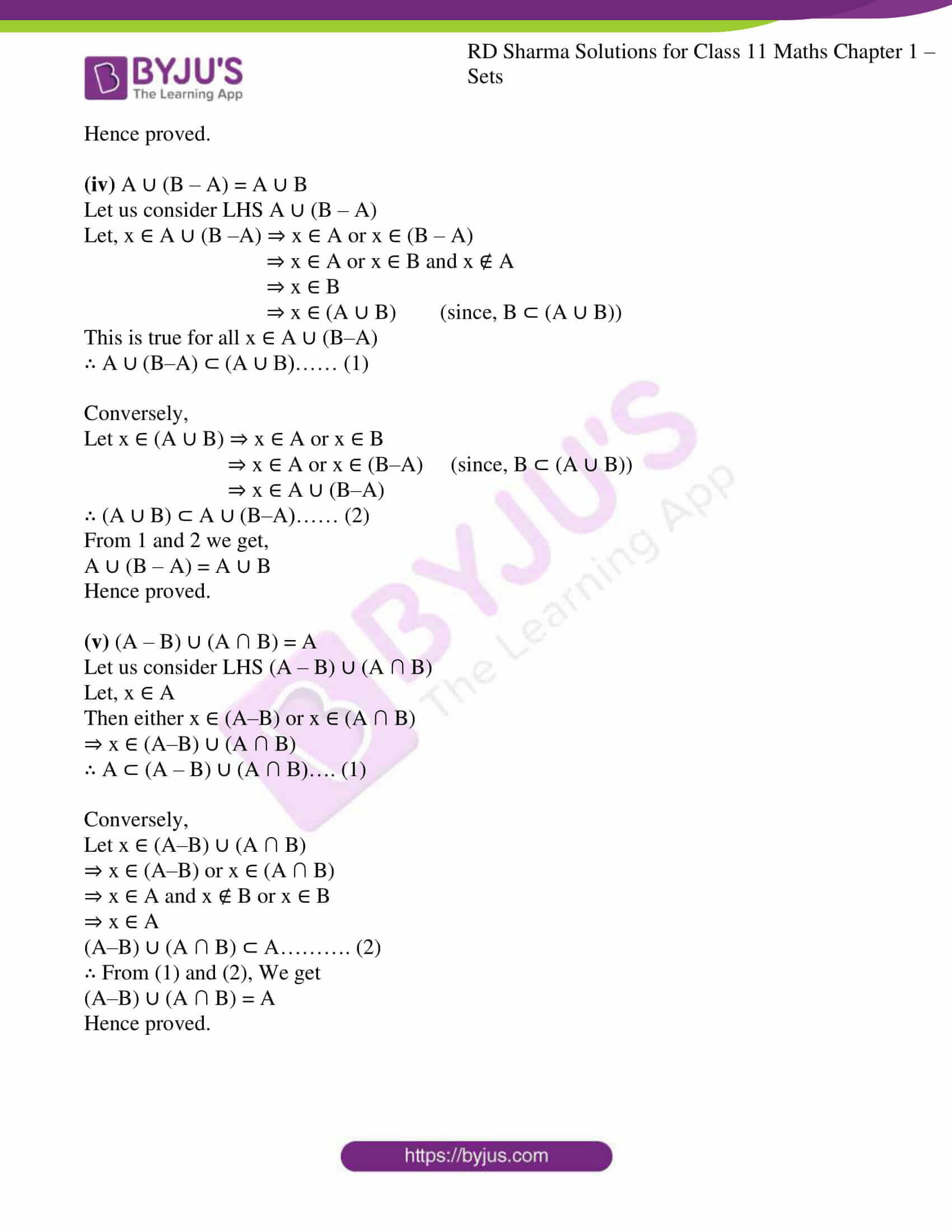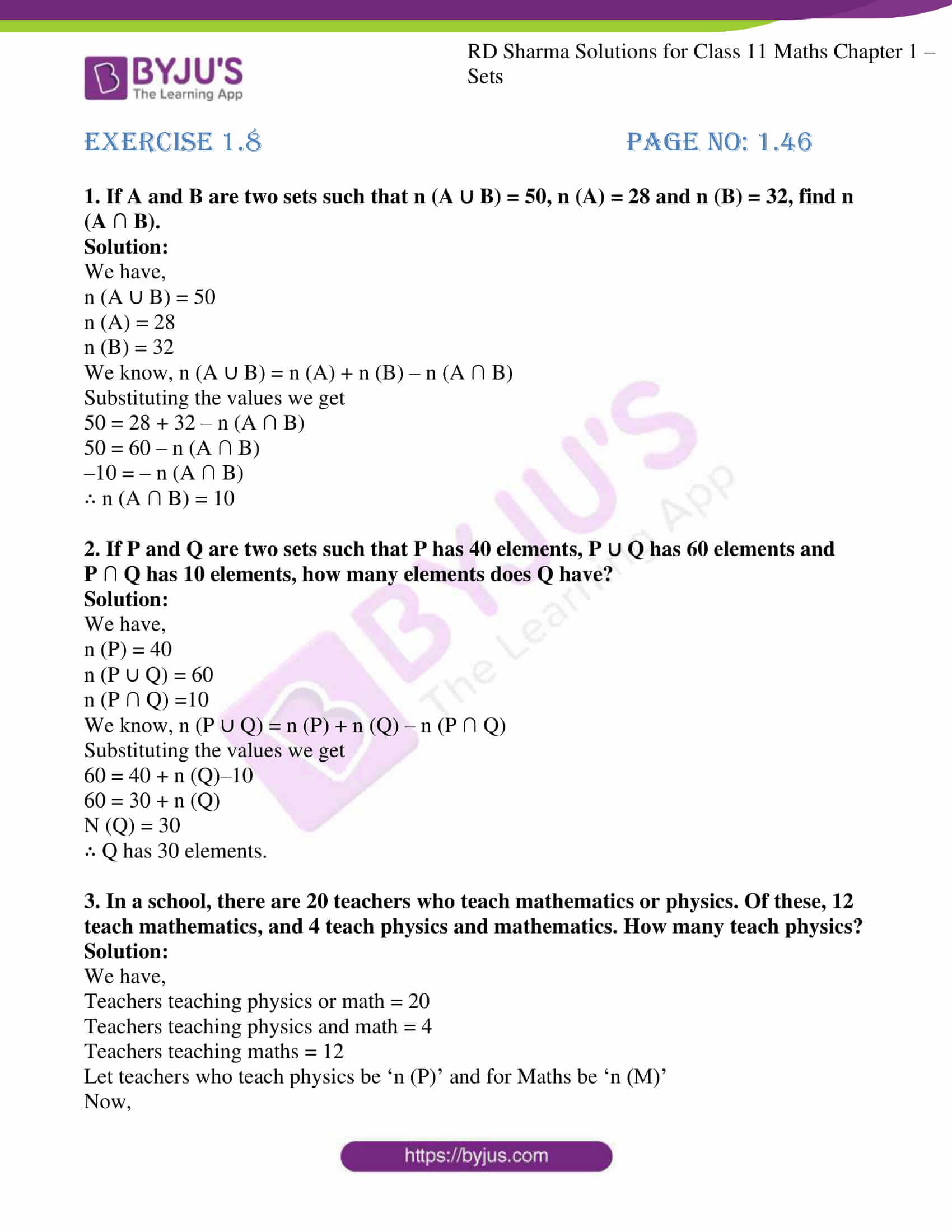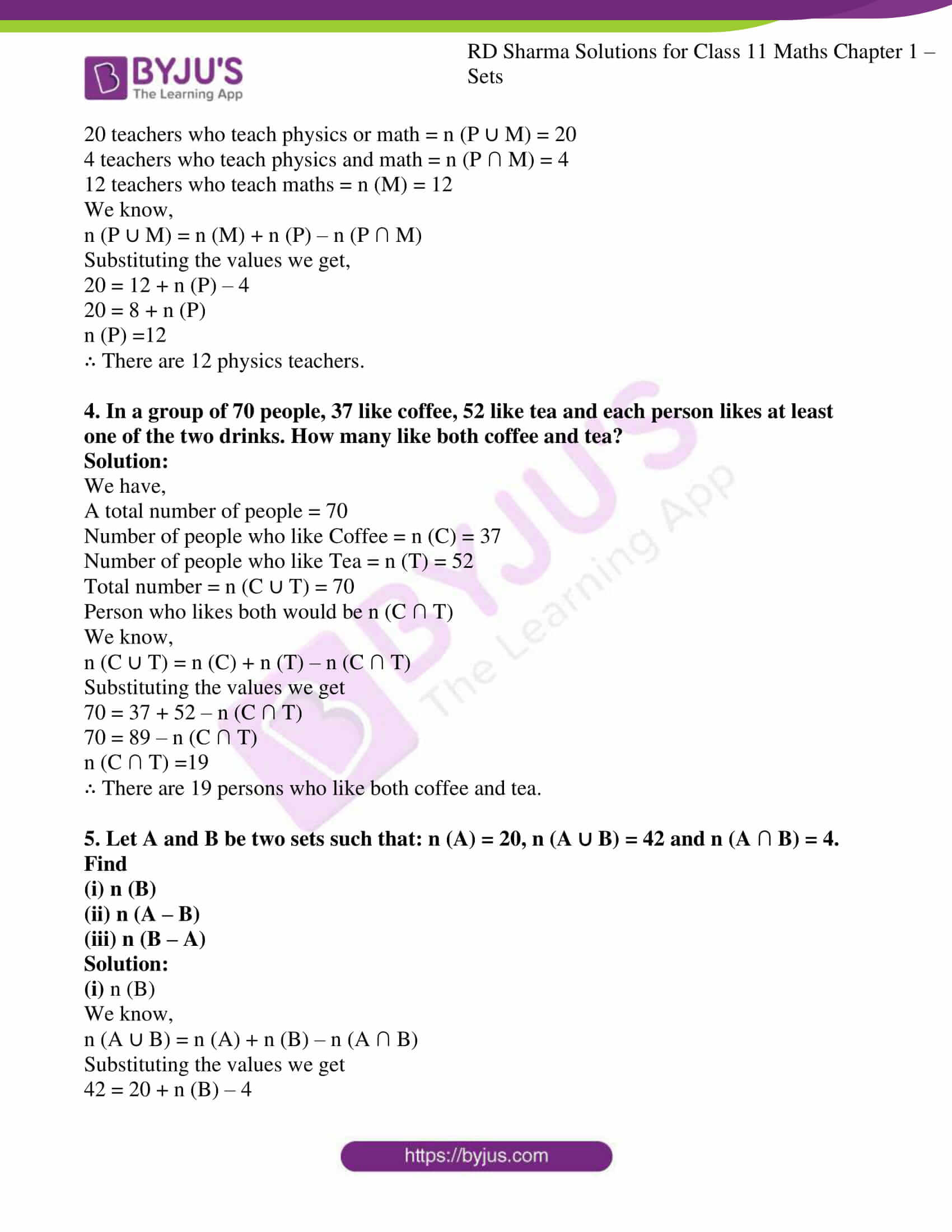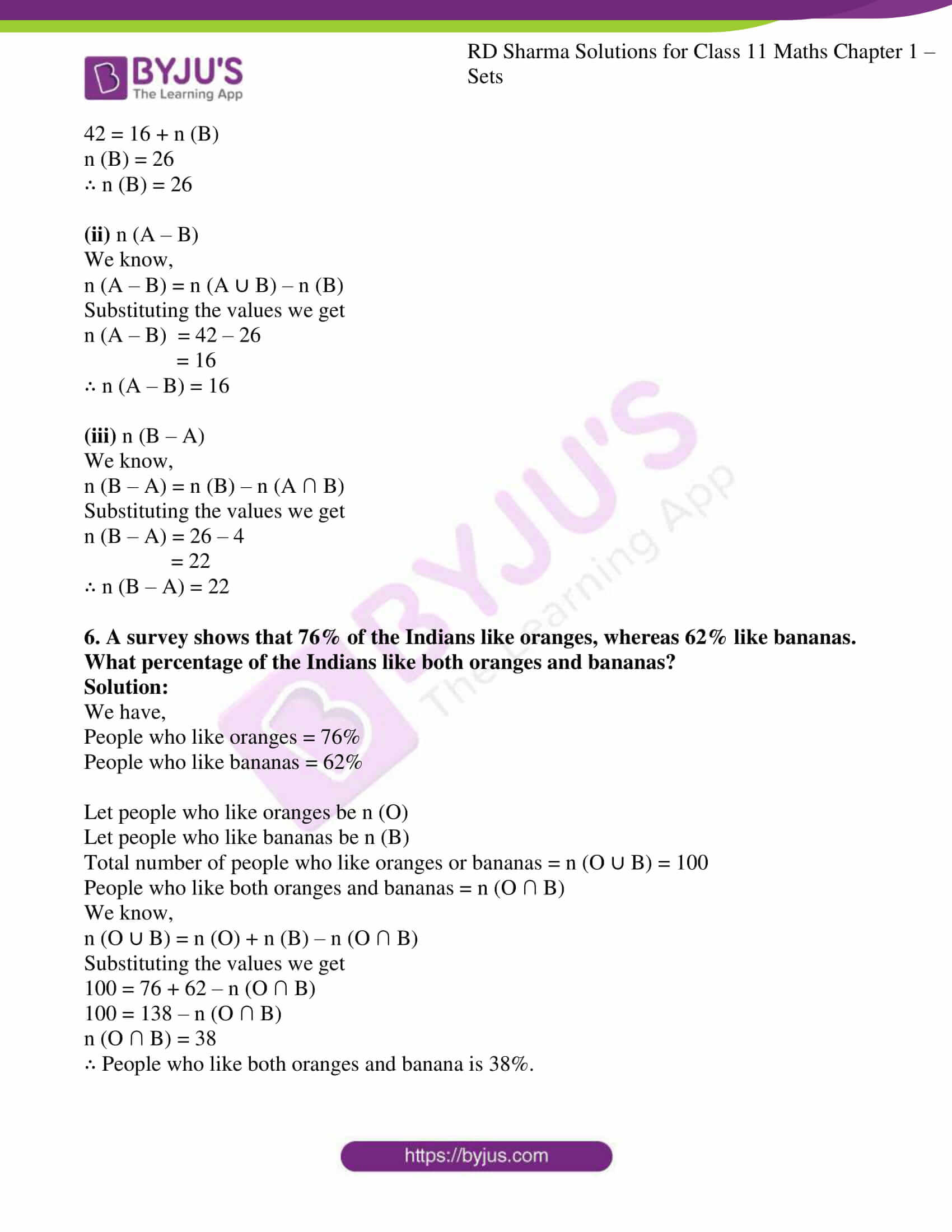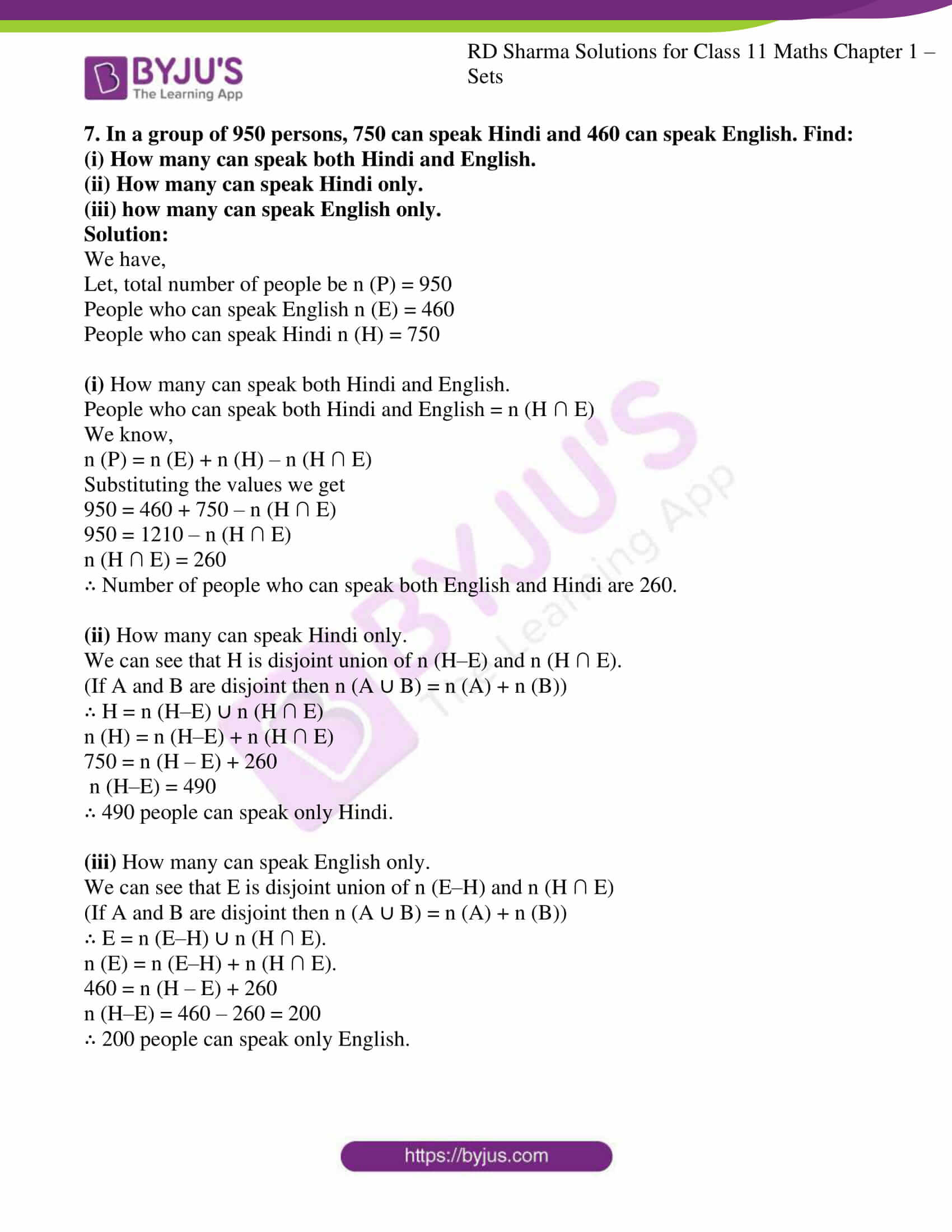### Access answers to RD Sharma Solutions for Class 11 Maths Chapter 1 – Sets

EXERCISE 1.1 PAGE NO: 1.2

1. What is the difference between a collection and a set? Give reasons to support your answer.

Solution:

Well defined collections are sets.

Examples: The collection of good cricket players of India is not a set. However, the collection of all good player is a set.

The collection of vowels in English alphabets is a set.

Thus, we can say that every set is a collection, but every collection is not necessarily a set. Only well defined collections are sets.

(i) A collection of all natural numbers less than 50.
(ii) The collection of good hockey players in India.
(iii) The collection of all the girls in your class.
(iv) The collection of all talented writers of India.
(v) The collection of difficult topics in Mathematics.
(vi) The collection of novels written by Munshi Prem Chand.
(vii) The collection of all questions of this chapter.
(viii) The collection of all months of a year beginning with the letter J.
(ix) A collection of most dangerous animals of the world.
(x) The collection of prime integers.

Solution:

(i) It is a set. Since, collection of all natural numbers less than 50 forms a set as it is well defined.

(ii) It is not a set. Since, the term ‘good’ is not well defined.

(iii) It is a set. Since, a collection of all the girls in your class it is a definite quantity hence it is a set.

(iv) It is not a set. Since, the term ‘most’ is not well defined. A writer may be talented in the eye of one person, but he may not be talented in the eye of some other person.

(v) It is not a set. Since, the term ‘difficult’ is not well defined. A topic may be difficult for one person, but may not be difficult for another person.

(vi) It is a set. Since, a collection of novels written by Munshi Prem Chand it is a definite quantity hence it is a set.

(vii) It is a set. Since, a collection of all the questions in this chapter. It is a definite quantity hence it is a set.

(viii) It is a set. Since, a collection of all months of a year beginning with the letter J. It is a definite quantity hence it is a set.

(ix) It is not a set. Since, the term ‘most dangerous’ is not well defined. The notion of dangerous animals differs from person to person.

(x) It is a set. Since, a collection of prime integers. It is a definite quantity hence it is a set.

3. If A={0,1,2,3,4,5,6,7,8,9,10}, then insert the appropriate symbol or in each of the following blank spaces:
(i) 4 ……A

(ii) -4……A
(iii) 12…..A

(iv) 9…..A
(v) 0……A

(vi) -2……A

Solution:

The symbol ‘∈’ means belongs to. ‘∉’ means does not belong to.

(i) 4 ……A

4 ∈ A (since, 4 is present in set A)

(ii) -4……A

-4 ∉ A (since, -4 is not present in set A)

(iii) 12…..A

12 ∉ A (since, 12 is not present in set A)

(iv) 9…..A

9 ∈ A (since, 9 is present in set A)

(v) 0……A

0 ∈ A (since, 0 is present in set A)

(vi) -2……A

-2 ∉ A (since, -2 is not present in set A)

EXERCISE 1.2 PAGE NO: 1.6

1. Describe the following sets in Roster form:

(i) {x : x is a letter before e in the English alphabet}

(ii) {x ∈ N: x2 < 25}

(iii) {x ∈ N: x is a prime number, 10 < x < 20}

(iv) {x ∈ N: x = 2n, n ∈ N}

(v) {x ∈ R: x > x}

(vi) {x : x is a prime number which is a divisor of 60}

(vii) {x : x is a two digit number such that the sum of its digits is 8}

(viii) The set of all letters in the word ‘Trigonometry’

(ix) The set of all letters in the word ‘Better.’

Solution:

(i) {x : x is a letter before e in the English alphabet}

So, when we read whole sentence it becomes x is such that x is a letter before ‘e’ in the English alphabet. Now letters before ‘e’ are a,b,c,d.

∴ Roster form will be {a,b,c,d}.

(ii) {x ∈ N: x2 < 25}

x ∈ N that implies x is a natural number.

x2 < 25

x < ±5

As x belongs to the natural number that means x < 5.

All numbers less than 5 are 1,2,3,4.

∴ Roster form will be {1,2,3,4}.

(iii) {x ∈ N: x is a prime number, 10 < x < 20}

X is a natural number and is between 10 and 20.

X is such that X is a prime number between 10 and 20.

Prime numbers between 10 and 20 are 11,13,17,19.

∴ Roster form will be {11,13,17,19}.

(iv) {x ∈ N: x = 2n, n ∈ N}

X is a natural number also x = 2n

∴ Roster form will be {2,4,6,8…..}.

This an infinite set.

(v) {x ∈ R: x > x}

Any real number is equal to its value it is neither less nor greater.

So, Roster form of such real numbers which has value less than itself has no such numbers.

∴ Roster form will be ϕ. This is called a null set.

(vi) {x : x is a prime number which is a divisor of 60}

All numbers which are divisor of 60 are = 1,2,3,4,5,6,10,12,15,20,30,60.

Now, prime numbers are = 2, 3, 5.

∴ Roster form will be {2, 3, 5}.

(vii) {x : x is a two digit number such that the sum of its digits is 8}

Numbers which have sum of its digits as 8 are = 17, 26, 35, 44, 53, 62, 71, 80

∴ Roster form will be {17, 26, 35, 44, 53, 62, 71, 80}.

(viii) The set of all letters in the word ‘Trigonometry’

As repetition is not allowed in a set, then the distinct letters are

Trigonometry = t, r, i, g, o, n, m, e, y

∴ Roster form will be {t, r, i, g, o, n, m, e, y}

(ix) The set of all letters in the word ‘Better.’

As repetition is not allowed in a set, then the distinct letters are

Better = b, e, t, r

∴ Roster form will be {b, e, t, r}

2. Describe the following sets in set-builder form:
(i) A = {1, 2, 3, 4, 5, 6}
(ii) B = {1, 1/2, 1/3, 1/4, 1/5, …..}
(iii) C = {0, 3, 6, 9, 12,….}
(iv) D = {10, 11, 12, 13, 14, 15}
(v) E = {0}
(vi) {1, 4, 9, 16,…,100}
(vii) {2, 4, 6, 8,….}
(viii) {5, 25, 125, 625}

Solution:

(i) A = {1, 2, 3, 4, 5, 6}

{x : x ∈ N, x<7}

This is read as x is such that x belongs to natural number and x is less than 7. It satisfies all condition of roster form.

(ii) B = {1, 1/2, 1/3, 1/4, 1/5, …}

{x : x = 1/n, n ∈ N}

This is read as x is such that x =1/n, where n ∈ N.

(iii) C = {0, 3, 6, 9, 12, ….}

{x : x = 3n, n ∈ Z+, the set of positive integers}

This is read as x is such that C is the set of multiples of 3 including 0.

(iv) D = {10, 11, 12, 13, 14, 15}

{x : x ∈ N, 9<x<16}

This is read as x is such that D is the set of natural numbers which are more than 9 but less than 16.

(v) E = {0}

{x : x = 0}

This is read as x is such that E is an integer equal to 0.

(vi) {1, 4, 9, 16,…, 100}

Where,

12 = 1

22 = 4

32 = 9

42 = 16

.

.

.

102 = 100

So, above set can be expressed in set-builder form as {x2: x ∈ N, 1≤ x ≤10}

(vii) {2, 4, 6, 8,….}

{x: x = 2n, n ∈ N}

This is read as x is such that the given set are multiples of 2.

(viii) {5, 25, 125, 625}

Where,

51 = 5

52 = 25

53 = 125

54 = 625

So, above set can be expressed in set-builder form as {5n: n ∈ N, 1≤ n ≤ 4}

3. List all the elements of the following sets:

(i) A={x : x2≤ 10, x Z}

(ii) B = {x : x = 1/(2n-1), 1 ≤ n ≤ 5}

(iii) C = {x : x is an integer, -1/2 < x < 9/2}

(iv) D={x : x is a vowel in the word “EQUATION”}

(v) E = {x : x is a month of a year not having 31 days}

(vi) F={x : x is a letter of the word “MISSISSIPPI”}

Solution:

(i) A={x : x2≤ 10, x ∈ Z}

First of all, x is an integer hence it can be positive and negative also.

x2 ≤ 10

(-3)2 = 9 < 10

(-2)2 = 4 < 10

(-1)2 = 1 < 10

02 = 0 < 10

12 = 1 < 10

22 = 4 < 10

32 = 9 < 10

Square root of next integers are greater than 10.

x ≤ √10

x = 0, ±1, ±2, ±3

A = {0, ±1, ±2, ±3}

(ii) B = {x : x = 1/(2n-1), 1 ≤ n ≤ 5}

Let us substitute the value of n to find the values of x.

At n=1, x = 1/(2(1)-1) = 1/1

At n=2, x = 1/(2(2)-1) = 1/3

At n=3, x = 1/(2(3)-1) = 1/5

At n=4, x = 1/(2(4)-1) = 1/7

At n=5, x = 1/(2(5)-1) = 1/9

x = 1, 1/3, 1/5, 1/7, 1/9

∴ B = {1, 1/3, 1/5, 1/7, 1/9}

(iii) C = {x : x is an integer, -1/2 < x < 9/2}

Given, x is an integer between -1/2 and 9/2

So all integers between -0.5<x<4.5 are = 0, 1, 2, 3, 4

∴ C = {0, 1, 2, 3, 4}

(iv) D={x : x is a vowel in the word “EQUATION”}

All vowels in the word ‘EQUATION’ are E, U, A, I, O

∴ D = {A, E, I, O, U}

(v) E = {x : x is a month of a year not having 31 days}

A month has either 28, 29, 30, 31 days.

Out of 12 months in a year which are not having 31 days are:

February, April, June, September, November.

∴ E: {February, April, June, September, November}

(vi) F = {x : x is a letter of the word “MISSISSIPPI”}

Letters in word ‘MISSISSIPPI’ are M, I, S, P.

∴ F = {M, I, S, P}.

4. Match each of the sets on the left in the roster form with the same set on the right described in the set-builder form:
(i) {A,P,L,E} (i) {x : x+5=5, x
z}
(ii) {5,-5} (ii) {x : x is a prime natural number and a divisor of 10}
(iii) {0} (iii) {x : x is a letter of the word “RAJASTHAN”}
(iv) {1, 2, 5, 10} (iv) {x : x is a natural and divisor of 10}
(v) {A, H, J, R, S, T, N} (v) {x : x2 – 25 =0}
(vi) {2,5} (vi) {x : x is a letter of word “APPLE”}

Solution:

(i) {A, P, L, E}{x: x is a letter of word “APPLE”}

(ii) {5,-5} {x: x2 – 25 =0}

The solution set of x2 – 25 = 0 is x = ±5

(iii) {0}{x: x+5=5, x ∈ z}

The solution set of x + 5 = 5 is x = 0.

(iv) {1, 2, 5, 10} {x: x is a natural and divisor of 10}

The natural numbers which are divisor of 10 are 1, 2, 5, 10.

(v) {A, H, J, R, S, T, N} {x: x is a letter of the word “RAJASTHAN”}

The distinct letters of word “RAJASTHAN” are A, H, J, R, S, T, N.

(vi) {2, 5} {x: x is a prime natural number and a divisor of 10}

The prime natural numbers which are divisor of 10 are 2, 5.

5. Write the set of all vowels in the English alphabet which precede q.

Solution:

Set of all vowels which precede q are

A, E, I, O these are the vowels they come before q.

∴ B = {A, E, I, O}.

6. Write the set of all positive integers whose cube is odd.

Solution:

Every odd number has an odd cube

Odd numbers can be represented as 2n+1.

{2n+1: n ∈ Z, n>0} or

{1,3,5,7,……}

7. Write the set {1/2, 2/5, 3/10, 4/17, 5/26, 6/37, 7/50} in the set-builder form.

Solution:

Where,

2 = 12 + 1

5 = 22 + 1

10 = 32 + 1

.

.

50 = 72 + 1

Here we can see denominator is square of numerator +1.

So, we can write the set builder form as

{n/(n2+1): n ∈ N, 1≤ n≤ 7}

EXERCISE 1.3 PAGE NO: 1.9

1. Which of the following are examples of empty set?
(i) Set of all even natural numbers divisible by 5.
(ii) Set of all even prime numbers.
(iii) {x: x2–2=0 and x is rational}.
(iv) {x: x is a natural number, x < 8 and simultaneously x > 12}.
(v) {x: x is a point common to any two parallel lines}.

Solution:

(i) All numbers ending with 0. Except 0 is divisible by 5 and are even natural number. Hence it is not an example of empty set.

(ii) 2 is a prime number and is even, and it is the only prime which is even. So this not an example of the empty set.

(iii) x2 – 2 = 0, x2 = 2, x = ± √2 ∈ N. There is not natural number whose square is 2. So it is an example of empty set.

(iv) There is no natural number less than 8 and greater than 12. Hence it is an example of the empty set.

(v) No two parallel lines intersect at each other. Hence it is an example of empty set.

2. Which of the following sets are finite and which are infinite?
(i) Set of concentric circles in a plane.
(ii) Set of letters of the English Alphabets.
(iii) {x ∈ N: x > 5}
(iv) {x ∈ N: x < 200}
(v) {x ∈ Z: x < 5}
(vi) {x ∈ R: 0 < x < 1}.

Solution:

(i) Infinite concentric circles can be drawn in a plane. Hence it is an infinite set.

(ii) There are just 26 letters in English Alphabets. Hence it is finite set.

(iii) It is an infinite set because, natural numbers greater than 5 is infinite.

(iv) It is a finite set. Since, natural numbers start from 1 and there are 199 numbers less than 200. Hence it is a finite set.

(v) It is an infinite set. Because integers less than 5 are infinite so it is an infinite set.

(vi) It is an infinite set. Because between two real numbers, there are infinite real numbers.

3. Which of the following sets are equal?
(i) A = {1, 2, 3}
(ii) B = {x ∈ R:x2–2x+1=0}
(iii) C = (1, 2, 2, 3}
(iv) D = {x ∈ R : x3 – 6x2+11x – 6 = 0}.

Solution:

A set is said to be equal with another set if all elements of both the sets are equal and same.

A = {1, 2, 3}

B ={x ∈ R: x2–2x+1=0}

x2–2x+1 = 0

(x–1)2 = 0

∴ x = 1.

B = {1}

C= {1, 2, 2, 3}

In sets we do not repeat elements hence C can be written as {1, 2, 3}

D = {x ∈ R: x3 – 6x2+11x – 6 = 0}

For x = 1, x2–2x+1=0

= (1)3–6(1)2+11(1)–6

= 1–6+11–6

= 0

For x =2,

= (2)3–6(2)2+11(2)–6

= 8–24+22–6

= 0

For x =3,

= (3)3–6(3)2+11(3)–6

= 27–54+33–6

= 0

∴ D = {1, 2, 3}

Hence, the set A, C and D are equal.

4. Are the following sets equal?
A={x: x is a letter in the word reap},

B={x: x is a letter in the word paper},
C={x: x is a letter in the word rope}.

Solution:

For A

Letters in word reap

A ={R, E, A, P} = {A, E, P, R}

For B

Letters in word paper

B = {P, A, E, R} = {A, E, P, R}

For C

Letters in word rope

C = {R, O, P, E} = {E, O, P, R}.

Set A = Set B

Because every element of set A is present in set B

But Set C is not equal to either of them because all elements are not present.

5. From the sets given below, pair the equivalent sets:
A= {1, 2, 3}, B = {t, p, q, r, s}, C = {α, β, γ}, D = {a, e, i, o, u}.

Solution:

Equivalent set are different from equal sets, Equivalent sets are those which have equal number of elements they do not have to be same.

A = {1, 2, 3}

Number of elements = 3

B = {t, p, q, r, s}

Number of elements = 5

C = {α, β, γ}

Number of elements = 3

D = {a, e, i, o, u}

Number of elements = 5

∴ Set A is equivalent with Set C.

Set B is equivalent with Set D.

6. Are the following pairs of sets equal? Give reasons.
(i) A = {2, 3}, B = {x: x is a solution of x2 + 5x + 6= 0}

(ii) A={x: x is a letter of the word “WOLF”}
B={x: x is letter of word “FOLLOW”}

Solution:

(i) A = {2, 3}

B = x2 + 5x + 6 = 0

x2 + 3x + 2x + 6 = 0

x(x+3) + 2(x+3) = 0

(x+3) (x+2) = 0

x = -2 and -3

= {–2, –3}

Since, A and B do not have exactly same elements hence they are not equal.

(ii) Every letter in WOLF

A = {W, O, L, F} = {F, L, O, W}

Every letter in FOLLOW

B = {F, O, L, W} = {F, L, O, W}

Since, A and B have same number of elements which are exactly same, hence they are equal sets.

7. From the sets given below, select equal sets and equivalent sets.
A = {0, a}, B = {1, 2, 3, 4}, C = {4, 8, 12},
D = {3, 1, 2, 4}, E = {1, 0}, F = {8, 4, 12},
G = {1, 5, 7, 11}, H = {a, b}

Solution:

A = {0, a}

B = {1, 2, 3, 4}

C = {4, 8, 12}

D = {3, 1, 2, 4} = {1, 2, 3, 4}

E = {1, 0}

F = {8, 4, 12} = {4, 8, 12}

G = {1, 5, 7, 11}

H = {a, b}

Equivalent sets:

i. A, E, H (all of them have exactly two elements in them)

ii. B, D, G (all of them have exactly four elements in them)

iii. C, F (all of them have exactly three elements in them)

Equal sets:

i. B, D (all of them have exactly the same elements, so they are equal)

ii. C, F (all of them have exactly the same elements, so they are equal)

8. Which of the following sets are equal?
A = {x: x ∈ N, x < 3}
B = {1, 2}, C= {3, 1}
D = {x: x ∈ N, x is odd, x < 5}
E = {1, 2, 1, 1}

F = {1, 1, 3}

Solution:

A = {1, 2}

B = {1, 2}

C = {3, 1}

D = {1, 3} (since, the odd natural numbers less than 5 are 1 and 3)

E = {1, 2} (since, repetition is not allowed)

F = {1, 3} (since, repetition is not allowed)

∴ Sets A, B and E are equal.

C, D and F are equal.

9. Show that the set of letters needed to spell “CATARACT” and the set of letters needed to spell “TRACT” are equal.

Solution:

For “CATARACT”

Distinct letters are

{C, A, T, R} = {A, C, R, T}

For “TRACT”

Distinct letters are

{T, R, A, C} = {A, C, R, T}

As we see letters need to spell cataract is equal to set of letters need to spell tract.

Hence the two sets are equal.

EXERCISE 1.4 PAGE NO: 1.16

1. Which of the following statements are true? Give a reason to support your answer.
(i) For any two sets A and B either A B or B A.
(ii) Every subset of an infinite set is infinite.
(iii) Every subset of a finite set is finite.
(iv) Every set has a proper subset.
(v) {a, b, a, b, a, b,….} is an infinite set.
(vi) {a, b, c} and {1, 2, 3} are equivalent sets.
(vii) A set can have infinitely many subsets.

Solution:

(i) False

No, it is not necessary for any two set A and B to be either A B or B A.

(ii) False

A = {1,2,3} It is finite subset of infinite set N of natural numbers.

(iii) True

Logically smaller part of something finite can never be infinite.

So, every subset of a finite set is finite.

(iv) False

Null set or empty set does not have a proper subset.

(v) False

We do not repeat elements in a set, so the given set becomes {a, b} which is a finite set.

(vi) True

Equivalent sets have same number of elements.

(vii) False

In A = {1}

The subsets can be ϕ and {1} which are finite.

2. State whether the following statements are true or false:
(i) 1 ∈ { 1,2,3}
(ii) a ⊂ {b,c,a}
(iii) {a} ∈ {a,b,c}
(iv) {a, b} = {a, a, b, b, a}
(v) The set {x: x + 8 = 8} is the null set.

Solution:

(i) True

1 belongs to the given set {1, 2, 3} as it is present in it.

(ii) False

Since, a is an element and not a subset of a set {b, c, a}

(iii) False

Since, {a} is a subset of set {b, c, a} and not an element.

(iv) True

We do not repeat same elements in a given set.

(v) False

Given, x+8 = 8

i.e. x = 0

So, the given set is a singleton set {0}. Where it is not a null set.

3. Decide among the following sets, which are subsets of which:
A = {x: x satisfies x2 – 8x + 12=0}, B = {2,4,6}, C = {2,4,6,8,….}, D = {6}

Solution:

A = x2 – 8x + 12=0

⇒ (x–6) (x–2) =0

⇒ x = 2 or x = 6

A = {2, 6}

B = {2, 4, 6}

C = {2, 4, 6, 8}

D = {6}

So we can say

D⊂A⊂B⊂C

4. Write which of the following statements are true? Justify your answer.
(i) The set of all integers is contained in the set of all rational numbers.
(ii) The set of all crows is contained in the set of all birds.
(iii) The set of all rectangles is contained in the set of all squares.
(iv) The set of all rectangle is contained in the set of all squares.
(v) The sets P = {a} and B = {{a}} are equal.
(vi) The sets A={x: x is a letter of word “LITTLE”} AND, b = {x: x is a letter of the word “TITLE”} are equal.

Solution:

(i) True

A rational number is represented by the form p/q where p and q are integers and (q not equal to 0) keeping q = 1 we can place any number as p. Which then will be an integer.

(ii) True

Crows are also birds, so they are contained in the set of all birds.

(iii) False

Every square can be a rectangle, but every rectangle cannot be a square.

(iv) False

Every square can be a rectangle, but every rectangle cannot be a square.

(v) False

P = {a}

B = {{a}}

But {a} = P

B = {P}

Hence they are not equal.

(vi) True

A = For “LITTLE”

A = {L, I, T, E} = {E, I, L, T}

B = For “TITLE”

B = {T, I, L, E} = {E, I, L, T}

∴ A = B

5. Which of the following statements are correct? Write a correct form of each of the incorrect statements.
(i) a ⊂ {a, b, c}
(ii) {a} {a, b, c}
(iii) a {{a}, b}
(iv) {a} ⊂ {{a}, b}
(v) {b, c} ⊂ {a,{b, c}}
(vi) {a, b} ⊂ {a,{b, c}}
(vii) ϕ {a, b}
(viii) ϕ ⊂ {a, b, c}
(ix) {x: x + 3 = 3}= ϕ

Solution:

(i) This isn’t subset of given set but belongs to the given set.

∴ The correct form would be

a ∈{a,b,c}

(ii) In this {a} is subset of {a, b, c}

∴ The correct form would be

{a} ⊂ {a, b, c}

(iii) ‘a’ is not the element of the set.

∴ The correct form would be

{a} ∈ {{a}, b}

(iv) {a} is not a subset of given set.

∴ The correct form would be

{a} ∈ {{a}, b}

(v) {b, c} is not a subset of given set. But it belongs to the given set.

∴ The correct form would be

{b, c} ∈ {a,{b, c}}

(vi) {a, b} is not a subset of given set.

∴ The correct form would be

{a, b}⊄{a,{b, c}}

(vii) ϕ does not belong to the given set but it is subset.

∴ The correct form would be

ϕ ⊂ {a, b}

(viii) It is the correct form. ϕ is subset of every set.

(ix) x + 3 = 3

x = 0 = {0}

It is not ϕ

∴ The correct form would be

{x: x + 3 = 3} ≠ ϕ

6. Let A = {a, b,{c, d}, e}. Which of the following statements are false and why?
(i) {c, d} ⊂ A
(ii) {c, d} A
(iii) {{c, d}} ⊂ A
(iv) a A
(v) a ⊂ A.
(vi) {a, b, e} ⊂ A
(vii) {a, b, e} A
(viii) {a, b, c} ⊂ A
(ix) ϕ A
(x) {ϕ} ⊂ A

Solution:

(i) False

{c, d} is not a subset of A but it belong to A.

{c, d} ∈ A

(ii) True

{c, d} ∈ A

(iii) True

{c, d} is a subset of A.

(iv) It is true that a belongs to A.

(v) False

a is not a subset of A but it belongs to A

(vi) True

{a, b, e} is a subset of A.

(vii) False

{a, b, e} does not belong to A, {a, b, e} ⊂ A this is the correct form.

(viii) False

{a, b, c} is not a subset of A

(ix) False

ϕ is a subset of A.

ϕ ⊂ A.

(x) False

{ϕ} is not subset of A, ϕ is a subset of A.

7. Let A = {{1, 2, 3}, {4, 5}, {6, 7, 8}}. Determine which of the following is true or false:
(i) 1 ∈ A
(ii) {1, 2, 3} ⊂ A
(iii) {6, 7, 8} ∈ A
(iv) {4, 5} ⊂ A
(v) ϕ ∈ A
(vi) ϕ ⊂ A

Solution:

(i) False

1 is not an element of A.

(ii) True

{1,2,3} ∈ A. this is correct form.

(iii) True.

{6, 7, 8} ∈ A.

(iv) True

{{4, 5}} is a subset of A.

(v) False

Φ is a subset of A, not an element of A.

(vi) True

Φ is a subset of every set, so it is a subset of A.

8. Let A = {ϕ, {ϕ}, 1, {1, ϕ}, 2}. Which of the following are true?
(i) ϕ ∈ A
(ii) {ϕ} ∈ A
(iii) {1} ∈ A
(iv) {2, ϕ} ⊂ A
(v) 2 ⊂ A
(vi) {2, {1}} ⊄A
(vii) {{2}, {1}} ⊄ A
(viii) {ϕ, {ϕ}, {1, ϕ}} ⊂ A
(ix) {{ϕ}} ⊂ A

Solution:

(i) True

Φ belongs to set A. Hence, true.

(ii) True

{Φ} is an element of set A. Hence, true.

(iii) False

1 is not an element of A. Hence, false.

(iv) True

{2, Φ} is a subset of A. Hence, true.

(v) False

2 is not a subset of set A, it is an element of set A. Hence, false.

(vi) True

{2, {1}} is not a subset of set A. Hence, true.

(vii) True

Neither {2} and nor {1} is a subset of set A. Hence, true.

(viii) True

All three {ϕ, {ϕ}, {1, ϕ}} are subset of set A. Hence, true.

(ix) True

{{ϕ}} is a subset of set A. Hence, true.

EXERCISE 1.5 PAGE NO: 1.21

1. If A and B are two sets such that A ⊂ B, then Find:
(i) A ⋂ B

(ii) A ⋃ B

Solution:

(i) A ∩ B

A ∩ B denotes A intersection B. Common elements of A and B consists in this set.

Given A ⊂ B, every element of A are already an element of B.

∴ A ∩ B = A

(ii) A ⋃ B

A ∪ B denotes A union B. Elements of either A or B or in both A and B consist in this set.

Given A ⊂ B, B is having all elements including elements of A.

∴ A ∪ B = B

2. If A = {1, 2, 3, 4, 5}, B = {4, 5, 6, 7, 8}, C = {7, 8, 9, 10, 11} and D = {10, 11, 12, 13, 14}. Find:
(i) A ∪ B
(ii) A ∪ C
(iii) B ∪ C
(iv) B ∪ D
(v) A ∪ B ∪ C
(vi) A ∪ B ∪ D
(vii) B ∪ C ∪ D
(viii) A ∩ (B ∪ C)
(ix) (A ∩ B) ∩ (B ∩ C)
(x) (A ∪ D) ∩ (B ∪ C).

Solution:

In general X ∪ Y = {a: a  X or a  Y}

X ∩ Y = {a: a  X and a  Y}.

(i) A = {1, 2, 3, 4, 5}

B = {4, 5, 6, 7, 8}

A ∪ B = {x: x  A or x  B}

= {1, 2, 3, 4, 5, 6, 7, 8}

(ii) A = {1, 2, 3, 4, 5}

C = {7, 8, 9, 10, 11}

A ∪ C = {x: x  A or x  C}

= {1, 2, 3, 4, 5, 7, 8, 9, 10, 11}

(iii) B = {4, 5, 6, 7, 8}

C = {7, 8, 9, 10, 11}

B ∪ C = {x: x  B or x  C}

= {4, 5, 6, 7, 8, 9, 10, 11}

(iv) B = {4, 5, 6, 7, 8}

D = {10, 11, 12, 13, 14}

B ∪ D = {x: x  B or x  D}

= {4, 5, 6, 7, 8, 10, 11, 12, 13, 14}

(v) A = {1, 2, 3, 4, 5}

B = {4, 5, 6, 7, 8}

C = {7, 8, 9, 10, 11}

A ∪ B = {x: x  A or x  B}

= {1, 2, 3, 4, 5, 6, 7, 8}

A ∪ B ∪ C = {x: x  A ∪ B or x  C}

= {1, 2, 3, 4, 5, 6, 7, 8, 9, 10, 11}

(vi) A = {1, 2, 3, 4, 5}

B = {4, 5, 6, 7, 8}

D = {10, 11, 12, 13, 14}

A ∪ B = {x: x  A or x  B}

= {1, 2, 3, 4, 5, 6, 7, 8}

A ∪ B ∪ D = {x: x  A ∪ B or x  D}

= {1, 2, 3, 4, 5, 6, 7, 8, 10, 11, 12, 13, 14}

(vii) B = {4, 5, 6, 7, 8}

C = {7, 8, 9, 10, 11}

D = {10, 11, 12, 13, 14}

B ∪ C = {x: x  B or x  C}

= {4, 5, 6, 7, 8, 9, 10, 11}

B ∪ C ∪ D = {x: x  B ∪ C or x  D}

= {4, 5, 6, 7, 8, 9, 10, 11, 12, 13, 14}

(viii) A = {1, 2, 3, 4, 5}

B = {4, 5, 6, 7, 8}

C = {7, 8, 9, 10, 11}

B ∪ C = {x: x  B or x  C}

= {4, 5, 6, 7, 8, 9, 10, 11}

A ∩ B ∪ C = {x: x  A and x  B ∪ C}

= {4, 5}

(ix) A = {1, 2, 3, 4, 5}

B = {4, 5, 6, 7, 8}

C = {7, 8, 9, 10, 11}

(A ∩ B) = {x: x  A and x  B}

= {4, 5}

(B ∩ C) = {x: x  B and x  C}

= {7, 8}

(A ∩ B) ∩ (B ∩ C) = {x: x  (A ∩ B) and x  (B ∩ C)}

= ϕ

(x) A = {1, 2, 3, 4, 5}

B = {4, 5, 6, 7, 8}

C = {7, 8, 9, 10, 11}

D = {10, 11, 12, 13, 14}

A ∪ D = {x: x  A or x  D}

= {1, 2, 3, 4, 5, 10, 11, 12, 13, 14}

B ∪ C = {x: x  B or x  C}

= {4, 5, 6, 7, 8, 9, 10, 11}

(A ∪ D) ∩ (B ∪ C) = {x: x  (A ∪ D) and x  (B ∪ C)}

= {4, 5, 10, 11}

3. Let A = {x: x ∈ N}, B = {x: x = 2n, n ∈ N), C = {x: x = 2n – 1, n ∈ N} and, D = {x: x is a prime natural number} Find:
(i) A ∩ B
(ii) A ∩ C
(iii) A ∩ D
(iv) B ∩ C
(v) B ∩ D
(vi) C ∩ D

Solution:

A = All natural numbers i.e. {1, 2, 3…..}

B = All even natural numbers i.e. {2, 4, 6, 8…}

C = All odd natural numbers i.e. {1, 3, 5, 7……}

D = All prime natural numbers i.e. {1, 2, 3, 5, 7, 11, …}

(i) A ∩ B

A contains all elements of B.

∴ B ⊂ A = {2, 4, 6, 8…}

∴ A ∩ B = B

(ii) A ∩ C

A contains all elements of C.

∴ C ⊂ A = {1, 3, 5…}

∴ A ∩ C = C

(iii) A ∩ D

A contains all elements of D.

∴ D ⊂ A = {2, 3, 5, 7..}

∴ A ∩ D = D

(iv) B ∩ C

B ∩ C = ϕ

There is no natural number which is both even and odd at same time.

(v) B ∩ D

B ∩ D = 2

{2} is the only natural number which is even and a prime number.

(vi) C ∩ D

C ∩ D = {1, 3, 5, 7…}

= D – {2}

Every prime number is odd except {2}.

EXERCISE 1.6 PAGE NO: 1.27

1. Find the smallest set A such that A ∪ {1, 2} = {1, 2, 3, 5, 9}.

Solution:

A ∪ {1, 2} = {1, 2, 3, 5, 9}

Elements of A and {1, 2} together give us the result

So smallest set of A can be

A = {1, 2, 3, 5, 9} – {1, 2}

A = {3, 5, 9}

2. Let A = {1, 2, 4, 5} B = {2, 3, 5, 6} C = {4, 5, 6, 7}. Verify the following identities:
(i) A
(B ∩ C) = (A  B) ∩ (A  C)

(ii) A ∩ (B  C) = (A ∩ B)  (A ∩ C)

(iii) A ∩ (B – C) = (A ∩ B) – (A ∩ C)

(iv) A – (B ∪ C) = (A – B) ∩ (A – C)

(v) A – (B ∩ C) = (A – B)  (A – C)

(vi) A ∩ (B  C) = (A ∩ B) △ (A ∩ C)

Solution:

(i) A ∪ (B ∩ C) = (A ∪ B) ∩ (A ∪ C)

Firstly let us consider the LHS

(B ∩ C) = {x: x  B and x  C}

= {5, 6}

A ∪ (B ∩ C) = {x: x  A or x  (B ∩ C)}

= {1, 2, 4, 5, 6}

Now, RHS

(A ∪ B) = {x: x  A or x  B}

= {1, 2, 4, 5, 6}.

(A ∪ C) = {x: x  A or x  C}

= {1, 2, 4, 5, 6, 7}

(A ∪ B) ∩ (A ∪ C) = {x: x  (A ∪ B) and x  (A ∪ C)}

= {1, 2, 4, 5, 6}

∴ LHS = RHS

Hence Verified.

(ii) A ∩ (B ∪ C) = (A ∩ B) ∪ (A ∩ C)

Firstly let us consider the LHS

(B ∪ C) = {x: x  B or x  C}

= {2, 3, 4, 5, 6, 7}

(A ∩ (B ∪ C)) = {x: x  A and x  (B ∪ C)}

= {2, 4, 5}

Now, RHS

(A ∩ B) = {x: x  A and x  B}

= {2, 5}

(A ∩ C) = {x: x  A and x  C}

= {4, 5}

(A ∩ B) ∪ (A ∩ C) = {x: x  (A∩B) and x  (A∩C)}

= {2, 4, 5}

∴ LHS = RHS

Hence verified.

(iii) A ∩ (B – C) = (A ∩ B) – (A ∩ C)

B–C is defined as {x  B: x ∉ C}

B = {2, 3, 5, 6}

C = {4, 5, 6, 7}

B–C = {2, 3}

Firstly let us consider the LHS

(A ∩ (B – C)) = {x: x  A and x  (B – C)}

= {2}

Now, RHS

(A ∩ B) = {x: x  A and x  B}

= {2, 5}

(A ∩ C) = {x: x  A and x  C}

= {4, 5}

(A ∩ B) – (A ∩ C) is defined as {x  (A ∩ B): x ∉ (A ∩ C)}

= {2}

∴ LHS = RHS

Hence Verified.

(iv) A – (B ∪ C) = (A – B) ∩ (A – C)

Firstly let us consider the LHS

(B ∪ C) = {x: x  B or x  C}

= {2, 3, 4, 5, 6, 7}.

A – (B ∪ C) is defined as {x  A: x ∉ (B ∪ C)}

A = {1, 2, 4, 5}

(B ∪ C) = {2, 3, 4, 5, 6, 7}

A – (B ∪ C) = {1}

Now, RHS

(A – B)

A – B is defined as {x  A: x ∉ B}

A = {1, 2, 4, 5}

B = {2, 3, 5, 6}

A – B = {1, 4}

(A – C)

A – C is defined as {x  A: x ∉ C}

A = {1, 2, 4, 5}

C = {4, 5, 6, 7}

A – C = {1, 2}

(A – B) ∩ (A – C) = {x: x  (A – B) and x  (A – C)}.

= {1}

∴ LHS = RHS

Hence verified.

(v) A – (B ∩ C) = (A – B) ∪ (A – C)

Firstly let us consider the LHS

(B ∩ C) = {x: x  B and x  C}

= {5, 6}

A – (B ∩ C) is defined as {x  A: x ∉ (B ∩ C)}

A = {1, 2, 4, 5}

(B ∩ C) = {5, 6}

(A – (B ∩ C)) = {1, 2, 4}

Now, RHS

(A – B)

A – B is defined as {x  A: x ∉ B}

A = {1, 2, 4, 5}

B = {2, 3, 5, 6}

A–B = {1, 4}

(A – C)

A – C is defined as {x  A: x ∉ C}

A = {1, 2, 4, 5}

C = {4, 5, 6, 7}

A – C = {1, 2}

(A – B) ∪ (A – C) = {x: x  (A – B) OR x  (A – C)}.

= {1, 2, 4}

∴ LHS = RHS

Hence verified.

(vi) A ∩ (B △ C) = (A ∩ B) △ (A ∩ C)

A = {1, 2, 4, 5} B = {2, 3, 5, 6} C = {4, 5, 6, 7}.

Firstly let us consider the LHS

A ∩ (B △ C)

B △ C = (B – C) ∪ (C – B) = {2, 3} ∪ {4, 7} = {2, 3, 4, 7}

A ∩ (B △ C) = {2, 4}

Now, RHS

A ∩ B = {2, 5}

A ∩ C = {4, 5}

(A ∩ B) △ (A ∩ C) = [(A ∩ B) – (A ∩ C)] ∪ [(A ∩ C) – (A ∩ B)]

= {2} ∪ {4}

= {2, 4}

∴ LHS = RHS

Hence, Verified.

3. If U = {2, 3, 5, 7, 9} is the universal set and A = {3, 7}, B = {2, 5, 7, 9}, then prove that:
(i) (A ∪ B)’ = A’ ∩ B’

(ii) (A ∩ B)’ = A’  B’

Solution:

(i) (A ∪ B)’ = A’ ∩ B’

Firstly let us consider the LHS

A ∪ B = {x: x  A or x  B}

= {2, 3, 5, 7, 9}

(A∪B)’ means Complement of (A∪B) with respect to universal set U.

So, (A∪B)’ = U – (A∪B)’

U – (A∪B)’ is defined as {x  U: x ∉ (A∪B)’}

U = {2, 3, 5, 7, 9}

(A∪B)’ = {2, 3, 5, 7, 9}

U – (A∪B)’ = ϕ

Now, RHS

A’ means Complement of A with respect to universal set U.

So, A’ = U – A

(U – A) is defined as {x  U: x ∉ A}

U = {2, 3, 5, 7, 9}

A = {3, 7}

A’ = U – A = {2, 5, 9}

B’ means Complement of B with respect to universal set U.

So, B’ = U – B

(U – B) is defined as {x  U: x ∉ B}

U = {2, 3, 5, 7, 9}

B = {2, 5, 7, 9}

B’ = U – B = {3}

A’ ∩ B’ = {x: x  A’ and x  C’}.

= ϕ

∴ LHS = RHS

Hence verified.

(ii) (A ∩ B)’ = A’ ∪ B’

Firstly let us consider the LHS

(A ∩ B)’

(A ∩ B) = {x: x  A and x  B}.

= {7}

(A∩B)’ means Complement of (A ∩ B) with respect to universal set U.

So, (A∩B)’ = U – (A ∩ B)

U – (A ∩ B) is defined as {x  U: x ∉ (A ∩ B)’}

U = {2, 3, 5, 7, 9}

(A ∩ B) = {7}

U – (A ∩ B) = {2, 3, 5, 9}

(A ∩ B)’ = {2, 3, 5, 9}

Now, RHS

A’ means Complement of A with respect to universal set U.

So, A’ = U – A

(U – A) is defined as {x  U: x ∉ A}

U = {2, 3, 5, 7, 9}

A = {3, 7}

A’ = U – A = {2, 5, 9}

B’ means Complement of B with respect to universal set U.

So, B’ = U – B

(U – B) is defined as {x  U: x ∉ B}

U = {2, 3, 5, 7, 9}

B = {2, 5, 7, 9}

B’ = U – B = {3}

A’ ∪ B’ = {x: x  A or x  B}

= {2, 3, 5, 9}

∴ LHS = RHS

Hence verified.

4. For any two sets A and B, prove that
(i) B ⊂ A ∪ B

(ii) A ∩ B ⊂ A

(iii) A ⊂ B ⇒ A ∩ B = A

Solution:

(i) B ⊂ A ∪ B

Let us consider an element ‘p’ such that it belongs to B

∴ p ∈ B

p ∈ B ∪ A

B ⊂ A ∪ B

(ii) A ∩ B ⊂ A

Let us consider an element ‘p’ such that it belongs to B

∴ p ∈ A ∩ B

p ∈ A and p ∈ B

A ∩ B ⊂ A

(iii) A ⊂ B ⇒ A ∩ B = A

Let us consider an element ‘p’ such that it belongs to A ⊂ B.

p ∈ A ⊂ B

Then, x ∈ B

Let and p ∈ A ∩ B

x ∈ A and x ∈ B

x ∈ A and x ∈ A (since, A ⊂ B)

∴ (A ∩ B) = A

5. For any two sets A and B, show that the following statements are equivalent:
(i) A ⊂ B

(ii) A – B = ϕ

(iii) A  B = B

(iv) A ∩ B = A

Solution:

(i) A ⊂ B

To show that the following four statements are equivalent, we need to prove (i)=(ii), (ii)=(iii), (iii)=(iv), (iv)=(v)

Firstly let us prove (i)=(ii)

We know, A–B = {x ∈ A: x ∉ B} as A ⊂ B,

So, Each element of A is an element of B,

∴ A–B = ϕ

Hence, (i)=(ii)

(ii) A – B = ϕ

We need to show that (ii)=(iii)

By assuming A–B = ϕ

To show: A∪B = B

∴ Every element of A is an element of B

So, A ⊂ B and so A∪B = B

Hence, (ii)=(iii)

(iii) A ∪ B = B

We need to show that (iii)=(iv)

By assuming A ∪ B = B

To show: A ∩ B = A.

∴ A⊂ B and so A ∩ B = A

Hence, (iii)=(iv)

(iv) A ∩ B = A

Finally, now we need to show (iv)=(i)

By assuming A ∩ B = A

To show: A ⊂ B

Since, A ∩ B = A, so A⊂B

Hence, (iv)=(i)

6. For three sets A, B, and C, show that
(i) A ∩ B = A ∩ C need not imply B = C.

(ii) A ⊂ B ⇒ C – B ⊂ C – A

Solution:

(i) A ∩ B = A ∩ C need not imply B = C.

Let us consider, A = {1, 2}

B = {2, 3}

C = {2, 4}

Then,

A ∩ B = {2}

A ∩ C = {2}

Hence, A ∩ B = A ∩ C, where, B is not equal to C

(ii) A ⊂ B ⇒ C – B ⊂ C – A

Given: A ⊂ B

To show: C–B ⊂ C–A

Let us consider x ∈ C– B

⇒ x ∈ C and x ∉ B [by definition C–B]

⇒ x ∈ C and x ∉ A

⇒ x ∈ C–A

Thus x ∈ C–B ⇒ x ∈ C–A. This is true for all x ∈ C–B.

∴ A ⊂ B ⇒ C – B ⊂ C – A

7. For any two sets, prove that:
(i) A ∪ (A ∩ B) = A

(ii) A ∩ (A ∪ B) = A

Solution:

(i) A ∪ (A ∩ B) = A

We know union is distributive over intersection

So, A ∪ (A ∩ B)

(A ∪ A) ∩ (A ∪ B) [Since, A ∪ A = A]

A ∩ (A ∪ B)

A

(ii) A ∩ (A ∪ B) = A

We know union is distributive over intersection

So, (A ∩ A) ∪ (A ∩ B)

A ∪ (A ∩ B) [Since, A ∩ A = A]

A

EXERCISE 1.7 PAGE NO: 1.34

1. For any two sets A and B, prove that: A – B = B – A

Solution:

To prove, A’ – B’ = B – A

Firstly we need to show

A’ – B’ ⊆ B – A

Let, x ∈ A’ – B’

⇒ x ∈ A’ and x ∉ B’

⇒ x ∉ A and x ∈ B (since, A ∩ A’ = ϕ )

⇒ x ∈ B – A

It is true for all x ∈ A’ – B’

∴ A’ – B’ = B – A

Hence Proved.

2. For any two sets A and B, prove the following:
(i) A ∩ (A
∪ B) = A ∩ B

(ii) A – (A – B) = A ∩ B

(iii) A ∩ (A ∪ B’) = ϕ

(iv) A – B = A Δ (A ∩ B)

Solution:

(i) A ∩ (A’ ∪ B) = A ∩ B

Let us consider LHS A ∩ (A’ ∪ B)

Expanding

(A ∩ A’) ∪ (A ∩ B)

We know, (A ∩ A’) =ϕ

⇒ ϕ ∪ (A∩ B)

⇒ (A ∩ B)

∴ LHS = RHS

Hence proved.

(ii) A – (A – B) = A ∩ B

For any sets A and B we have De-Morgan’s law

(A ∪ B)’ = A’ ∩ B’, (A ∩ B) ‘ = A’ ∪ B’

Consider LHS

= A – (A–B)

= A ∩ (A–B)’

= A ∩ (A∩B’)’

= A ∩ (A’ ∪ B’)’) (since, (B’)’ = B)

= A ∩ (A’ ∪ B)

= (A ∩ A’) ∪ (A ∩ B)

= ϕ ∪ (A ∩ B) (since, A ∩ A’ = ϕ)

= (A ∩ B) (since, ϕ ∪ x = x, for any set)

= RHS

∴ LHS=RHS

Hence proved.

(iii) A ∩ (A ∪ B’) = ϕ

Let us consider LHS A ∩ (A ∪ B’)

= A ∩ (A ∪ B’)

= A ∩ (A’∩ B’) (By De–Morgan’s law)

= (A ∩ A’) ∩ B’ (since, A ∩ A’ = ϕ)

= ϕ ∩ B’

= ϕ (since, ϕ ∩ B’ = ϕ)

= RHS

∴ LHS=RHS

Hence proved.

(iv) A – B = A Δ (A ∩ B)

Let us consider RHS A Δ (A ∩ B)

A Δ (A ∩ B) (since, E Δ F = (E–F) ∪ (F–E))

= (A – (A ∩ B)) ∪ (A ∩ B –A) (since, E – F = E ∩ F’)

= (A ∩ (A ∩ B)’) ∪ (A ∩ B ∩ A’)

= (A ∩ (A’ ∪ B’)) ∪ (A ∩ A’ ∩ B) (by using De-Morgan’s law and associative law)

= (A ∩ A’) ∪ (A ∩ B’) ∪ (ϕ ∩ B) (by using distributive law)

= ϕ ∪ (A ∩ B’) ∪ ϕ

= A ∩ B’ (since, A ∩ B’ = A–B)

= A – B

= LHS

∴ LHS=RHS

Hence Proved

3. If A, B, C are three sets such that A ⊂ B, then prove that C – B ⊂ C – A.

Solution:

Given, ACB

To prove: C – B ⊂ C – A

Let us consider, x ∈ C–B

⇒ x ∈ C and x ∉ B

⇒ x ∈ C and x ∉ A

⇒ x ∈ C – A

Thus, x ∈ C–B ⇒ x ∈ C – A

This is true for all x ∈ C–B

∴ C – B ⊂ C – A

Hence proved.

4. For any two sets A and B, prove that
(i) (A ∪ B) – B = A – B

(ii) A – (A ∩ B) = A – B

(iii) A – (A – B) = A ∩ B

(iv) A ∪ (B – A) = A ∪ B

(v) (A – B) ∪ (A ∩ B) = A

Solution:

(i) (A ∪ B) – B = A – B

Let us consider LHS (A ∪ B) – B

= (A–B) ∪ (B–B)

= (A–B) ∪ ϕ (since, B–B = ϕ)

= A–B (since, x ∪ ϕ = x for any set)

= RHS

Hence proved.

(ii) A – (A ∩ B) = A – B

Let us consider LHS A – (A ∩ B)

= (A–A) ∩ (A–B)

= ϕ ∩ (A – B) (since, A-A = ϕ)

= A – B

= RHS

Hence proved.

(iii) A – (A – B) = A ∩ B

Let us consider LHS A – (A – B)

Let, x ∈ A – (A–B) = x ∈ A and x ∉ (A–B)

x ∈ A and x ∉ (A ∩ B)

= x ∈ A ∩ (A ∩ B)

= x ∈ (A ∩ B)

= (A ∩ B)

= RHS

Hence proved.

(iv) A ∪ (B – A) = A ∪ B

Let us consider LHS A ∪ (B – A)

Let, x ∈ A ∪ (B –A) ⇒ x ∈ A or x ∈ (B – A)

⇒ x ∈ A or x ∈ B and x ∉ A

⇒ x ∈ B

⇒ x ∈ (A ∪ B) (since, B ⊂ (A ∪ B))

This is true for all x ∈ A ∪ (B–A)

∴ A ∪ (B–A) ⊂ (A ∪ B)…… (1)

Conversely,

Let x ∈ (A ∪ B) ⇒ x ∈ A or x ∈ B

⇒ x ∈ A or x ∈ (B–A) (since, B ⊂ (A ∪ B))

⇒ x ∈ A ∪ (B–A)

∴ (A ∪ B) ⊂ A ∪ (B–A)…… (2)

From 1 and 2 we get,

A ∪ (B – A) = A ∪ B

Hence proved.

(v) (A – B) ∪ (A ∩ B) = A

Let us consider LHS (A – B) ∪ (A ∩ B)

Let, x ∈ A

Then either x ∈ (A–B) or x ∈ (A ∩ B)

⇒ x ∈ (A–B) ∪ (A ∩ B)

∴ A ⊂ (A – B) ∪ (A ∩ B)…. (1)

Conversely,

Let x ∈ (A–B) ∪ (A ∩ B)

⇒ x ∈ (A–B) or x ∈ (A ∩ B)

⇒ x ∈ A and x ∉ B or x ∈ B

⇒ x ∈ A

(A–B) ∪ (A ∩ B) ⊂ A………. (2)

∴ From (1) and (2), We get

(A–B) ∪ (A ∩ B) = A

Hence proved.

EXERCISE 1.8 PAGE NO: 1.46

1. If A and B are two sets such that n (A ∪ B) = 50, n (A) = 28 and n (B) = 32, find n (A ∩ B).

Solution:

We have,

n (A ∪ B) = 50

n (A) = 28

n (B) = 32

We know, n (A ∪ B) = n (A) + n (B) – n (A ∩ B)

Substituting the values we get

50 = 28 + 32 – n (A ∩ B)

50 = 60 – n (A ∩ B)

–10 = – n (A ∩ B)

∴ n (A ∩ B) = 10

2. If P and Q are two sets such that P has 40 elements, P ∪ Q has 60 elements and P ∩ Q has 10 elements, how many elements does Q have?

Solution:

We have,

n (P) = 40

n (P ∪ Q) = 60

n (P ∩ Q) =10

We know, n (P ∪ Q) = n (P) + n (Q) – n (P ∩ Q)

Substituting the values we get

60 = 40 + n (Q)–10

60 = 30 + n (Q)

N (Q) = 30

∴ Q has 30 elements.

3. In a school, there are 20 teachers who teach mathematics or physics. Of these, 12 teach mathematics, and 4 teach physics and mathematics. How many teach physics?

Solution:

We have,

Teachers teaching physics or math = 20

Teachers teaching physics and math = 4

Teachers teaching maths = 12

Let teachers who teach physics be ‘n (P)’ and for Maths be ‘n (M)’

Now,

20 teachers who teach physics or math = n (P ∪ M) = 20

4 teachers who teach physics and math = n (P ∩ M) = 4

12 teachers who teach maths = n (M) = 12

We know,

n (P ∪ M) = n (M) + n (P) – n (P ∩ M)

Substituting the values we get,

20 = 12 + n (P) – 4

20 = 8 + n (P)

n (P) =12

∴ There are 12 physics teachers.

4. In a group of 70 people, 37 like coffee, 52 like tea and each person likes at least one of the two drinks. How many like both coffee and tea?

Solution:

We have,

A total number of people = 70

Number of people who like Coffee = n (C) = 37

Number of people who like Tea = n (T) = 52

Total number = n (C ∪ T) = 70

Person who likes both would be n (C ∩ T)

We know,

n (C ∪ T) = n (C) + n (T) – n (C ∩ T)

Substituting the values we get

70 = 37 + 52 – n (C ∩ T)

70 = 89 – n (C ∩ T)

n (C ∩ T) =19

∴ There are 19 persons who like both coffee and tea.

5. Let A and B be two sets such that: n (A) = 20, n (A ∪ B) = 42 and n (A ∩ B) = 4. Find

(i) n (B)

(ii) n (A – B)

(iii) n (B – A)

Solution:

(i) n (B)

We know,

n (A ∪ B) = n (A) + n (B) – n (A ∩ B)

Substituting the values we get

42 = 20 + n (B) – 4

42 = 16 + n (B)

n (B) = 26

∴ n (B) = 26

(ii) n (A – B)

We know,

n (A – B) = n (A ∪ B) – n (B)

Substituting the values we get

n (A – B) = 42 – 26

= 16

∴ n (A – B) = 16

(iii) n (B – A)

We know,

n (B – A) = n (B) – n (A ∩ B)

Substituting the values we get

n (B – A) = 26 – 4

= 22

∴ n (B – A) = 22

6. A survey shows that 76% of the Indians like oranges, whereas 62% like bananas. What percentage of the Indians like both oranges and bananas?

Solution:

We have,

People who like oranges = 76%

People who like bananas = 62%

Let people who like oranges be n (O)

Let people who like bananas be n (B)

Total number of people who like oranges or bananas = n (O ∪ B) = 100

People who like both oranges and bananas = n (O ∩ B)

We know,

n (O ∪ B) = n (O) + n (B) – n (O ∩ B)

Substituting the values we get

100 = 76 + 62 – n (O ∩ B)

100 = 138 – n (O ∩ B)

n (O ∩ B) = 38

∴ People who like both oranges and banana is 38%.

7. In a group of 950 persons, 750 can speak Hindi and 460 can speak English. Find:
(i) How many can speak both Hindi and English.
(ii) How many can speak Hindi only.
(iii) how many can speak English only.

Solution:

We have,

Let, total number of people be n (P) = 950

People who can speak English n (E) = 460

People who can speak Hindi n (H) = 750

(i) How many can speak both Hindi and English.

People who can speak both Hindi and English = n (H ∩ E)

We know,

n (P) = n (E) + n (H) – n (H ∩ E)

Substituting the values we get

950 = 460 + 750 – n (H ∩ E)

950 = 1210 – n (H ∩ E)

n (H ∩ E) = 260

∴ Number of people who can speak both English and Hindi are 260.

(ii) How many can speak Hindi only.

We can see that H is disjoint union of n (H–E) and n (H ∩ E).

(If A and B are disjoint then n (A ∪ B) = n (A) + n (B))

∴ H = n (H–E) ∪ n (H ∩ E)

n (H) = n (H–E) + n (H ∩ E)

750 = n (H – E) + 260

n (H–E) = 490

∴ 490 people can speak only Hindi.

(iii) How many can speak English only.

We can see that E is disjoint union of n (E–H) and n (H ∩ E)

(If A and B are disjoint then n (A ∪ B) = n (A) + n (B))

∴ E = n (E–H) ∪ n (H ∩ E).

n (E) = n (E–H) + n (H ∩ E).

460 = n (H – E) + 260

n (H–E) = 460 – 260 = 200

∴ 200 people can speak only English.

## Frequently Asked Questions on RD Sharma Solutions for Class 11 Maths Chapter 1

### How to start reading RD Sharma Solutions for Class 11 Maths Chapter 1?

Very first learn and understood sets definitions and functions by the help of BYJU’S website. Then go through the solutions provided by BYJU’S experts at their site. By solving these exercises one can be thorough in all the concepts present in RD Sharma Solutions for Class 11 Maths Chapter 1.

### What are the important topics from an exam perspective present in RD Sharma Solutions for Class 11 Maths Chapter 1?

The important topics present in RD Sharma Solutions for Class 11 Maths Chapter 1 are
Sets and Description of Set.
Roaster form or tabular form.
Set-builder form.
Types of sets.
Subsets, universal set, power set.
Venn diagrams.
Operations on sets.
Laws of the algebra of sets.
Some important results on the number of elements in sets.
By solving problems based on these concepts students can score well in Class 11 board exams.

### Is it necessary to learn all methods to solve sets of RD Sharma Solutions for Class 11 Maths Chapter 1?

Yes, it is compulsory to learn all methods to solve sets when different conditions are given of RD Sharma Solutions for Class 11 Maths Chapter 1. These topics will continue in higher studies as well as it may come in their finals of Class 11. These concepts are explained flawlessly by BYJU’S tutors. Hence, the main aim of these solutions formulated by BYJU’S experts is to provide knowledge on the fundamental aspects of Maths, which in turn, helps students to understand every concept clearly.